• 设计功能：1. 正弦信号绘制考虑到绘制的界面大小有限，所有信号统一绘制四个周期...2. 对两个正弦信号相加和相乘可以选择是加法型还是乘法型，红字代表当前的状态，fa1，A1是一信号的频率和幅度，fa2和A2是二信号的...
设计功能：1.  正弦信号绘制考虑到绘制的界面大小有限，所有信号统一绘制四个周期，如果是两个正弦相加或相乘，将会绘制频率小的四个周期，每个周期都会在x轴显示时间，单位秒，来表示不同频率的信号。即x(t)=Asin(2πfat)。检查时发现幅度显示有误，有机会会改。2.  对两个正弦信号相加和相乘可以选择是加法型还是乘法型，红字代表当前的状态，fa1，A1是一信号的频率和幅度，fa2和A2是二信号的频率和幅度。默认状态时，二信号的A2都是零。fa会自动取fa1和fa2中较小且不为零的值，来保证绘制的连续信号是频率小的四个周期。后续有机会可以加上相位φ；还可以加更多信号的功能，甚至可以单独为信号合成设计界面。当有一个信号幅度较小，频率较小，另一个信号幅度较大，频率较大，可以观测出明显的包络线现象。3.  对连续信号采样成离散信号。fs是采样频率。N是截取长度。对连续信号进行采样。x(n)=Asin(2πnfa/fs)。fs过小时，加法时，小于fa1和fa2中大的那个两倍时；乘法时，小于fa1和fa2乘积，且fa1，fa2都不为零时，会产生混叠现象；当N不是fs/fa的整数倍时会产生泄露现象，多个信号合成时，N不是任一fs/fan的整数倍时，都会产生泄露现象。由于界面有限，N过大时，大于一两千图就不清楚了，后续有机会可以单独为信号离散化设计界面。4.  对离散信号DFT，绘制频谱图，记录算法效率根据DFT公式，将其中的W写成e的指数形式，再展开成三角函数形式，将实部虚部分开存储，从而避免虚数j。X[n].Re=∑x(n)*cos(2πnm/N),0<=n<=N-1;X[n].Im=-∑x(n)*sin(2πnm/N),0<=n<=N-1。幅频图|X(n)|=(X[n].Re^2+X[n].Im^2)½,相频图φ[n]=atan(X[n].Im/X[n].Re)。5.  对离散信号FFT，绘制频谱图，记录算法效率根据FFT公式，同理。G[n].Re=∑x(2n)*cos(4πnm/N),0<=n<=N/2-1;G[n].Im=∑x(2n)*sin(4πnm/N),0<=n<=N/2-1;H[n].Re=∑x(2n+1)*cos(2π(2n+1)m/N),0<=n<=N/2-1;H[n].Im=∑x(2n+1)*sin(2π(2n+1)m/N),0<=n<=N/2-1;之后X[n].Re=G[n].Re+H[n].Re; X[n].Im=G[n].Im+H[n].Im; X[n+N/2].Re=G[n].Re-H[n].Re; X[n+N/2].Im=G[n].Im-H[n].Im。由于当N很小时，计算机计算时间很短，1ms不到，没有手段检测。一开始采取在最里层循环加延时1ms，这样大部分情况FFT都比DFT快，但强制延时会有不可抗的冲突，效率比一直会变，甚至有时DFT比FFT都快；所以又将这个延时改成了一个+1的计数，这样这个效率比是关于N的固定函数值。DFT和FFT在幅频图一致，但相频图有不一致的点，这些点的幅度都为零，我们暂时认为没有影响。6.  对DFT后的结果IDFT，绘制还原后的离散信号根据IDFT公式，用X[n]反求x[n]。x[n].Re=1/N*∑(X(n).Re*cos(2πnm/N)- X(n).Im*sin(2πnm/N)),0<=n<=N1;x[n].Im=1/N*∑(X(n).Im*sin(2πnm/N)+X(n).Re*sin(2πnm/N)),0<=n<=N-1；最后x[n]= (x[n].Re^2+x[n].Im^2)½。还原后的离散信号全为正值。后续有机会可以用内插函数还原成连续信号x(t)。算法过程：1.  采样算法：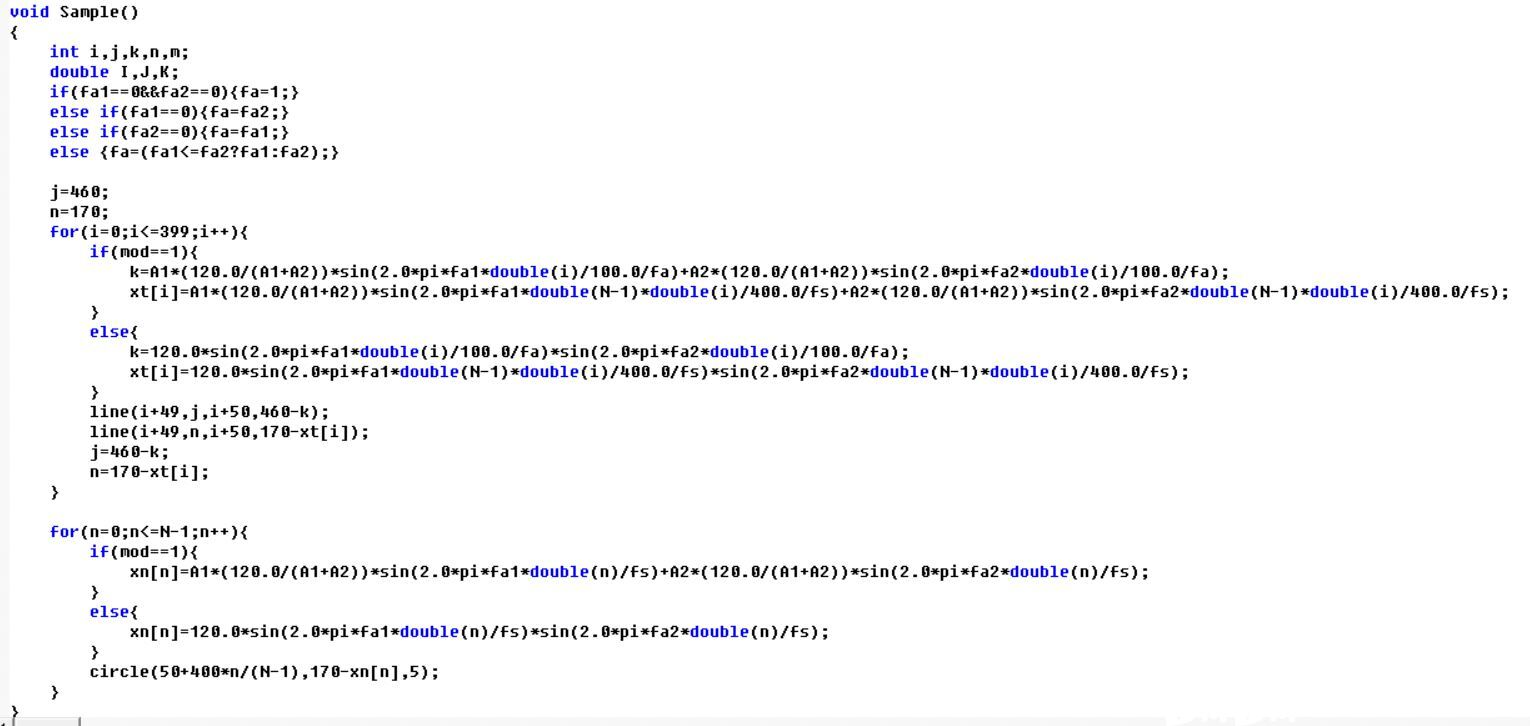2.  DFT算法：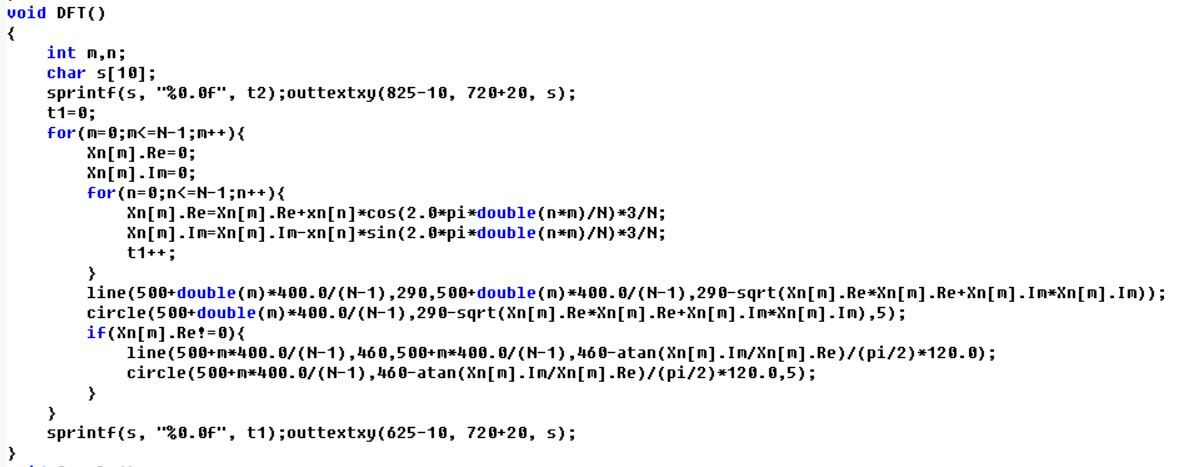3.  FFT算法：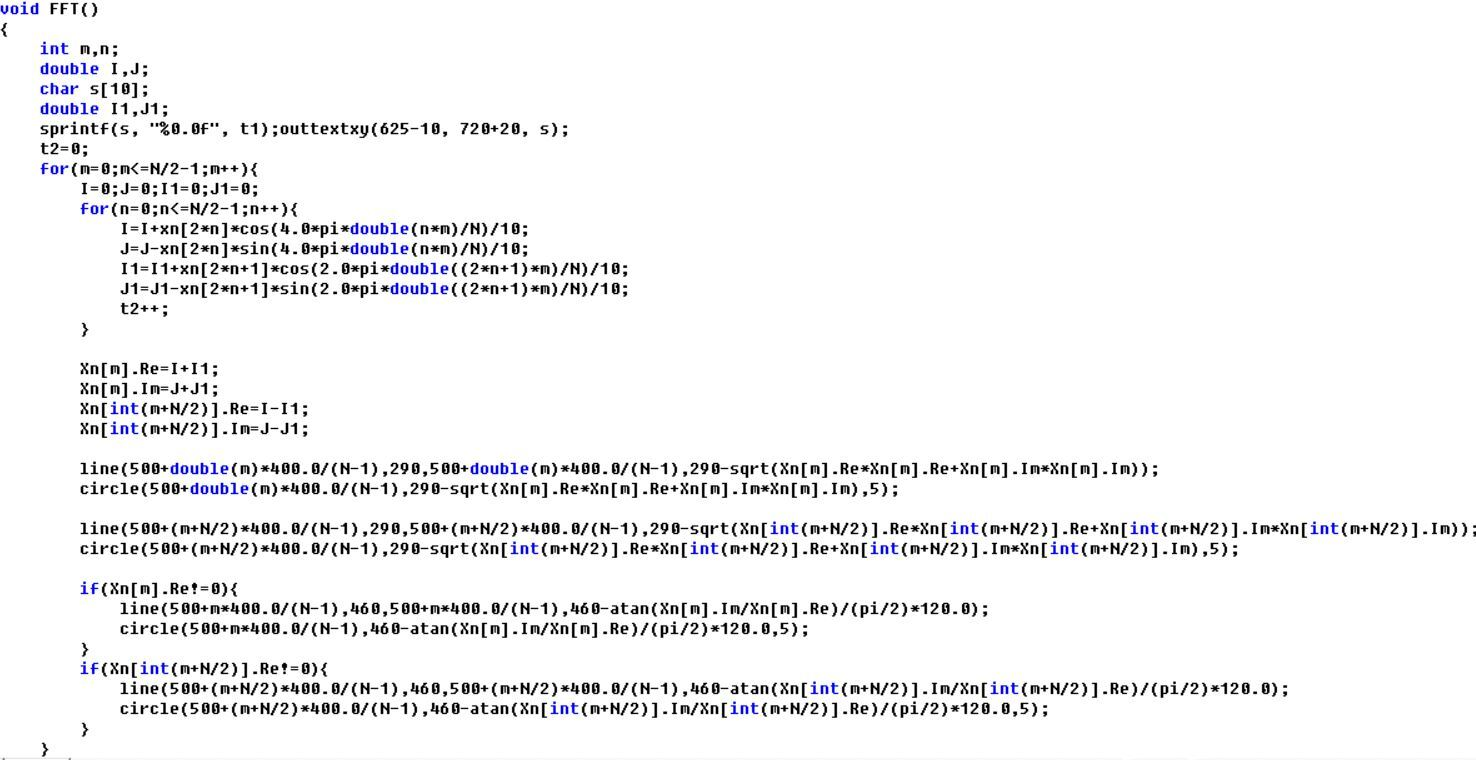4.  IDFT算法：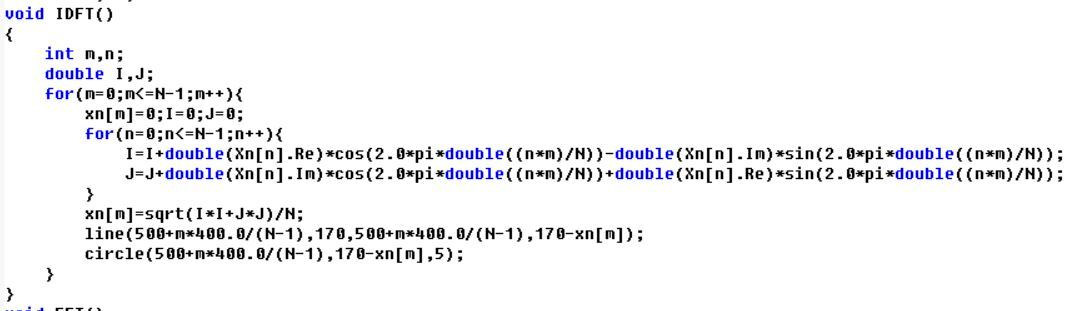演示结果：1.  不同单一频率正弦信号绘制：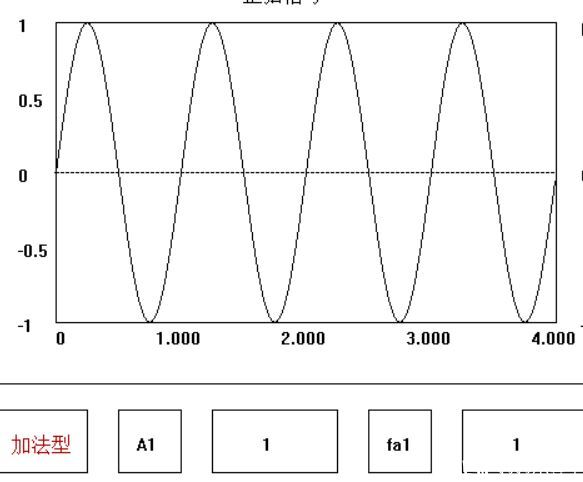上图为fa1=1HZ时。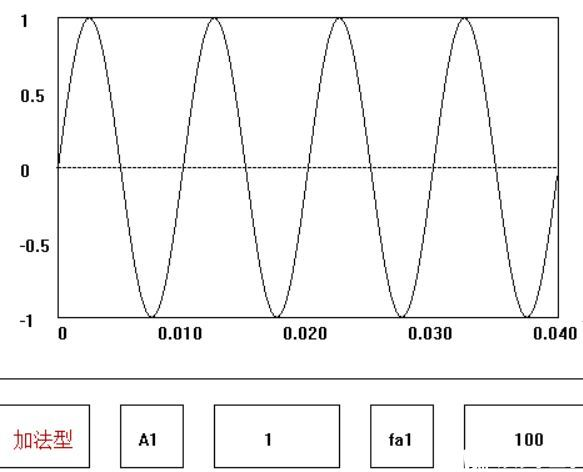上图为fa1=100HZ时。2.  正弦信号相加以及产生包络线：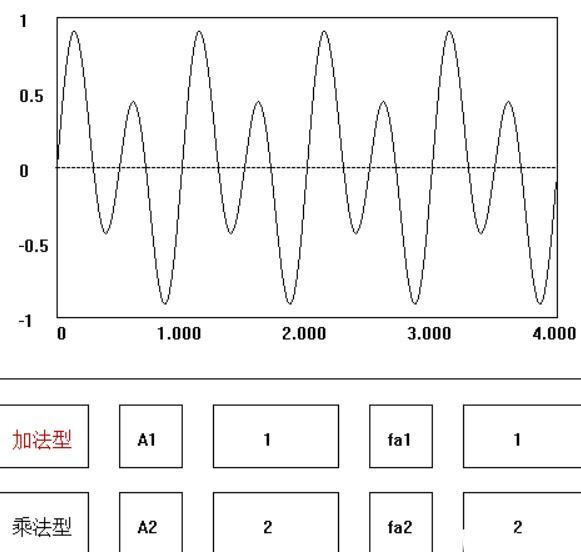上图是两个简单正弦波相加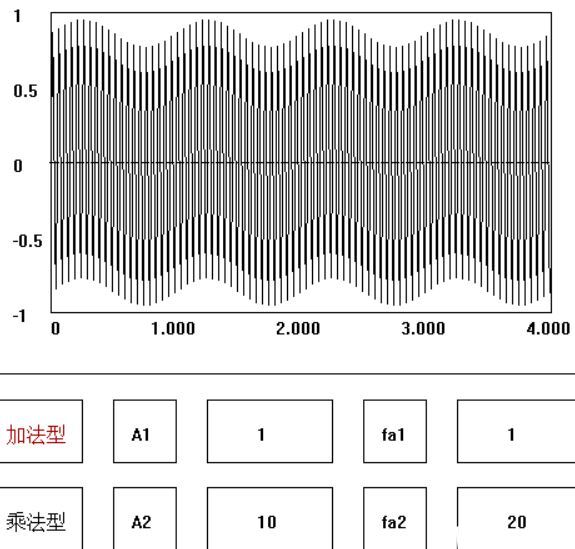上图是两个正弦波相加产生包络线(幅度相加，不同于高频学的频率相加，所以包络线不一样)3.  正弦信号相乘：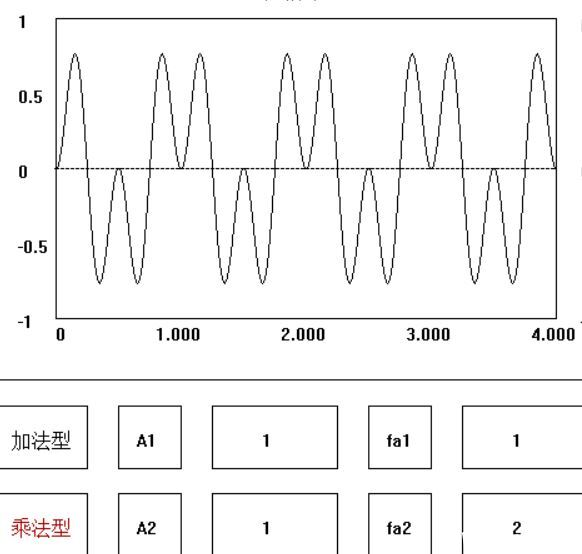上图是两个简单正弦波相乘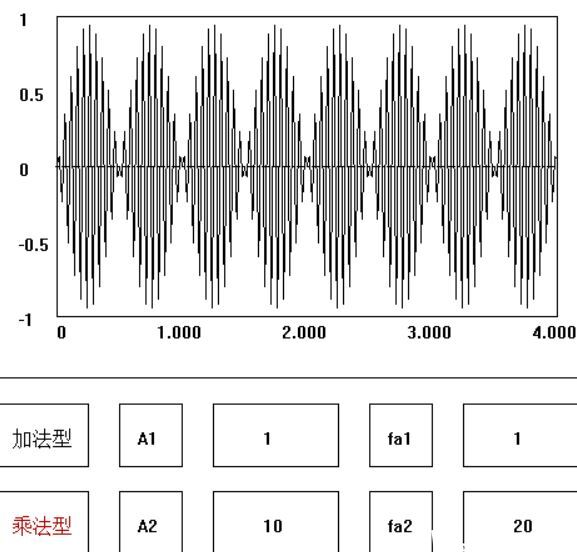上图是两个正弦波相乘(幅度相乘)4.  同一信号的不同采样频率采样：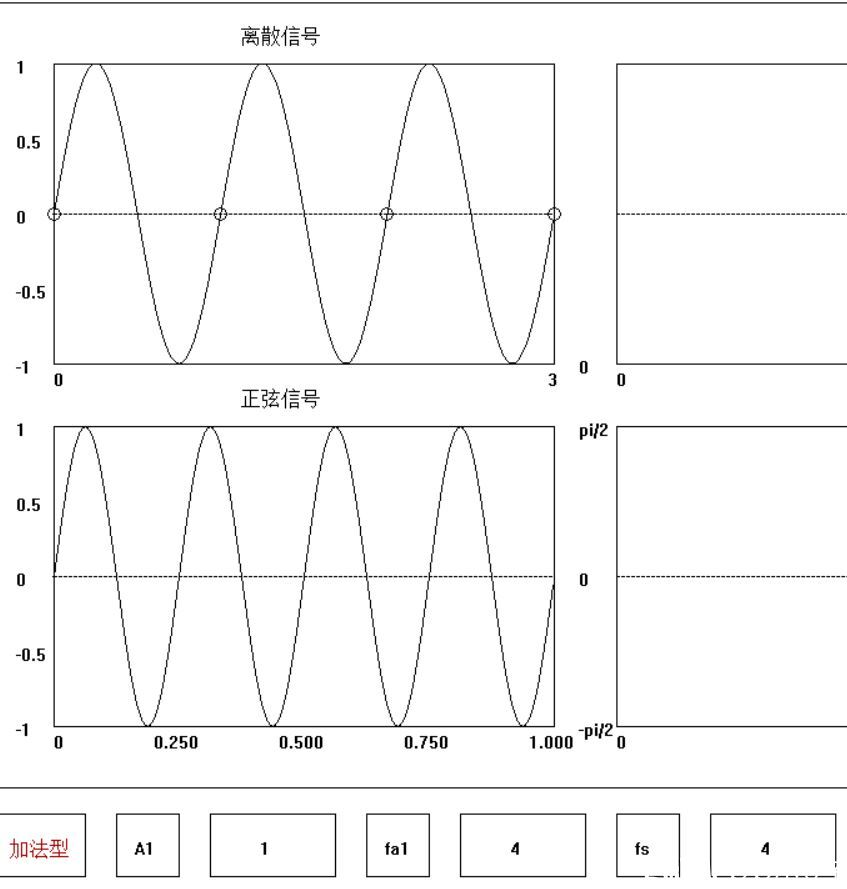上图是fs=fa时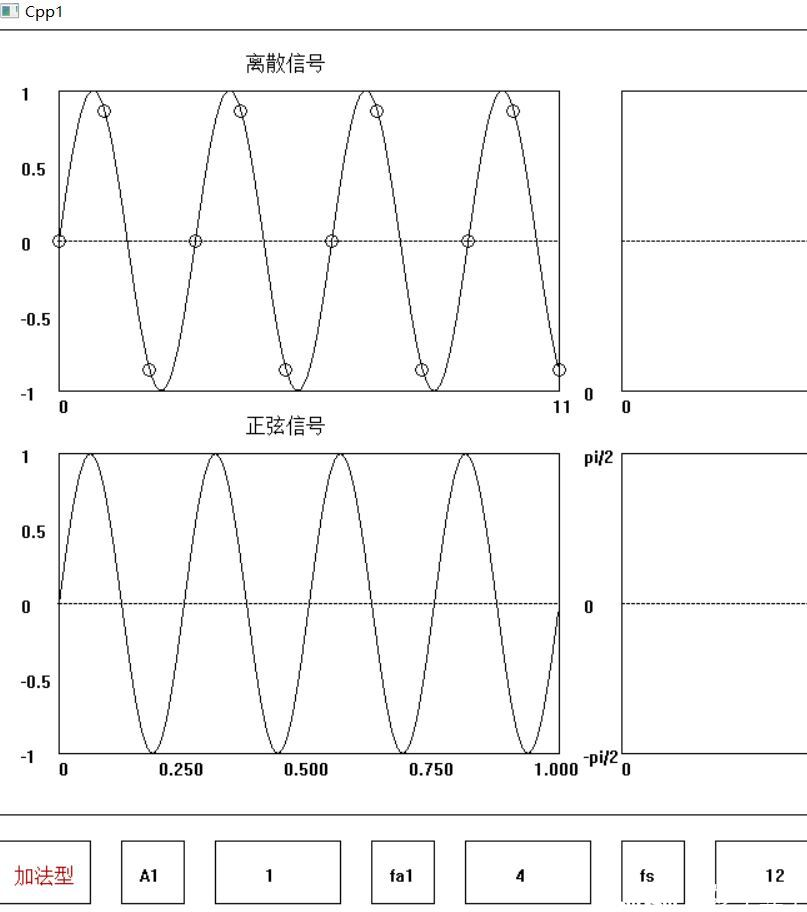上图是fs=3fa时5.  DFT、FFT效率(与N有关)：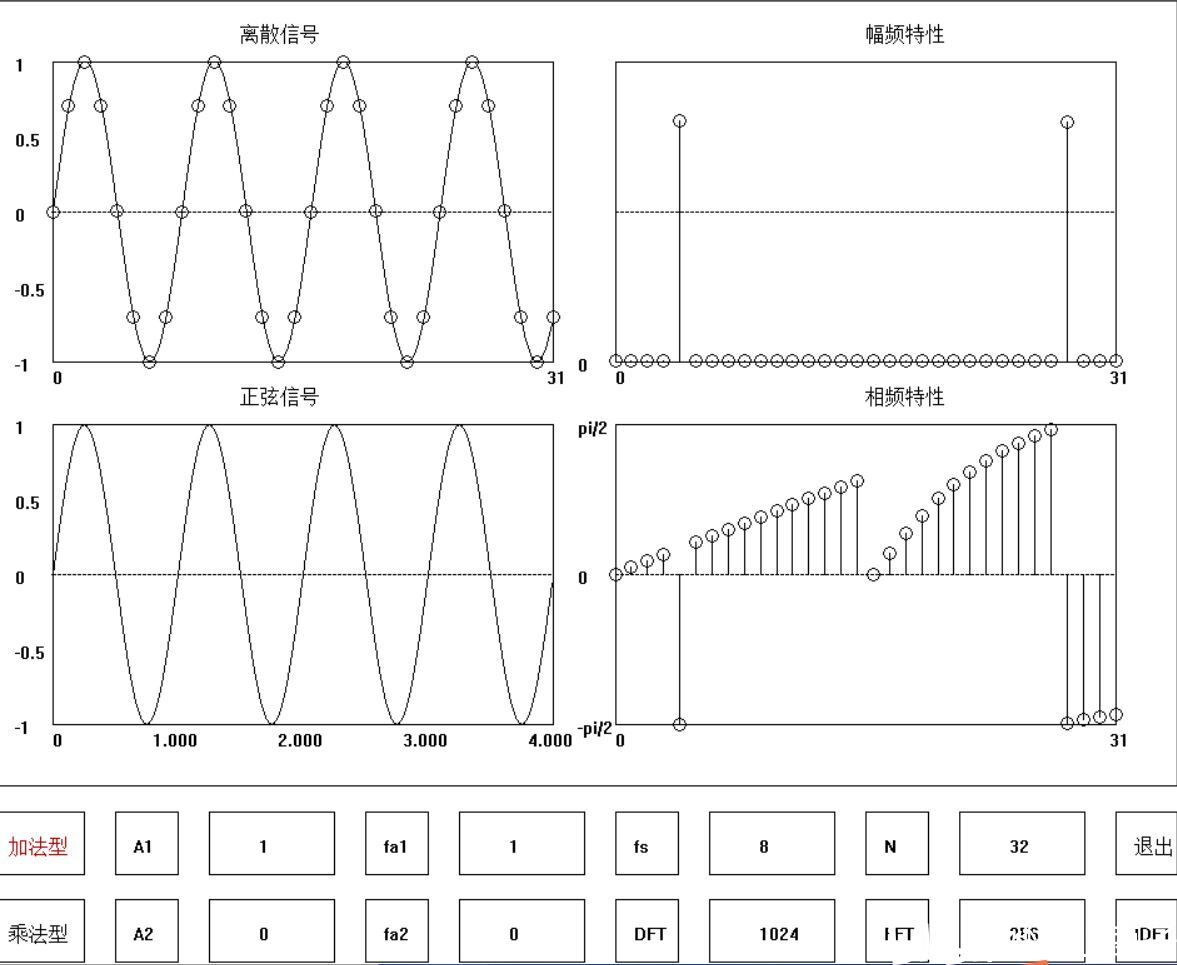上图是N=32时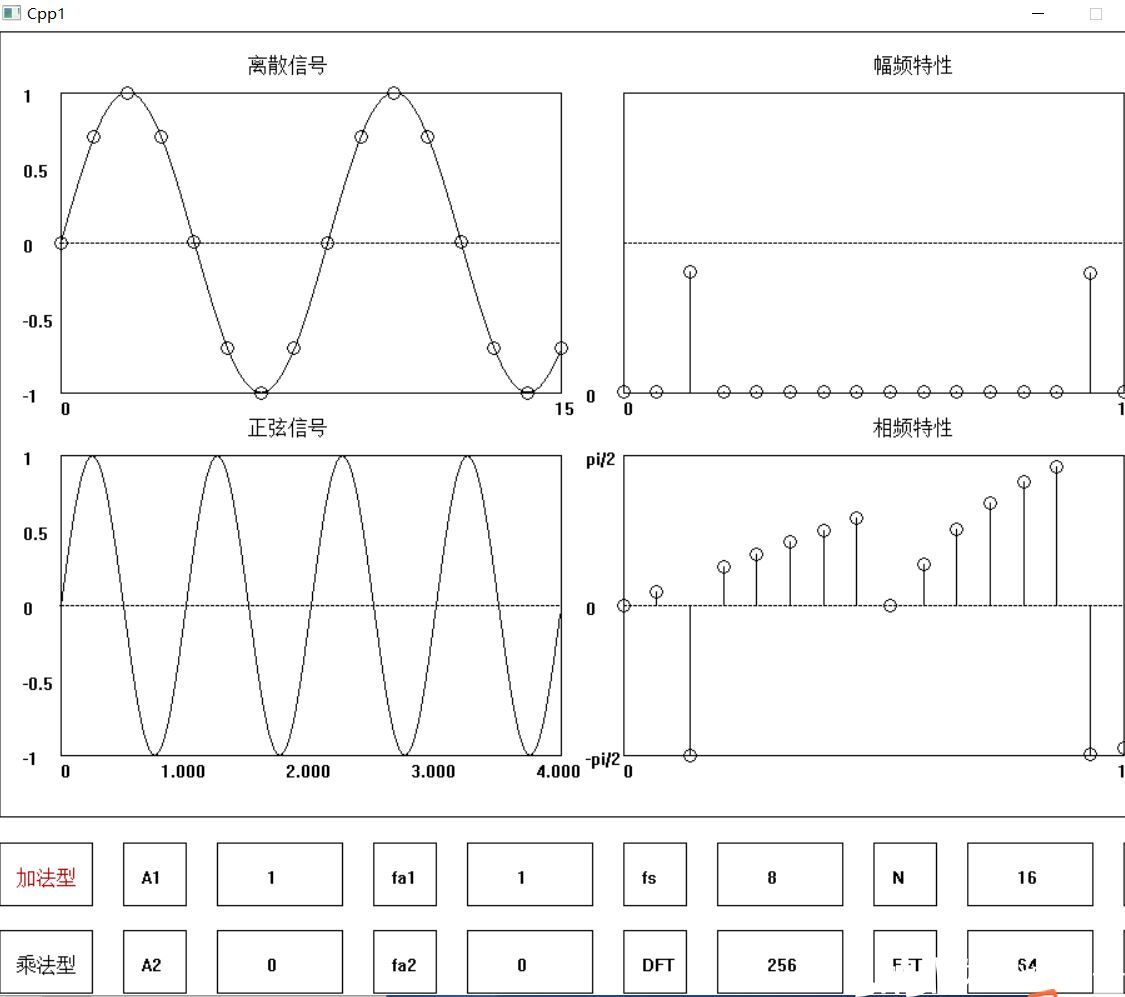上图是N=16时6.  混叠(fs<2fa)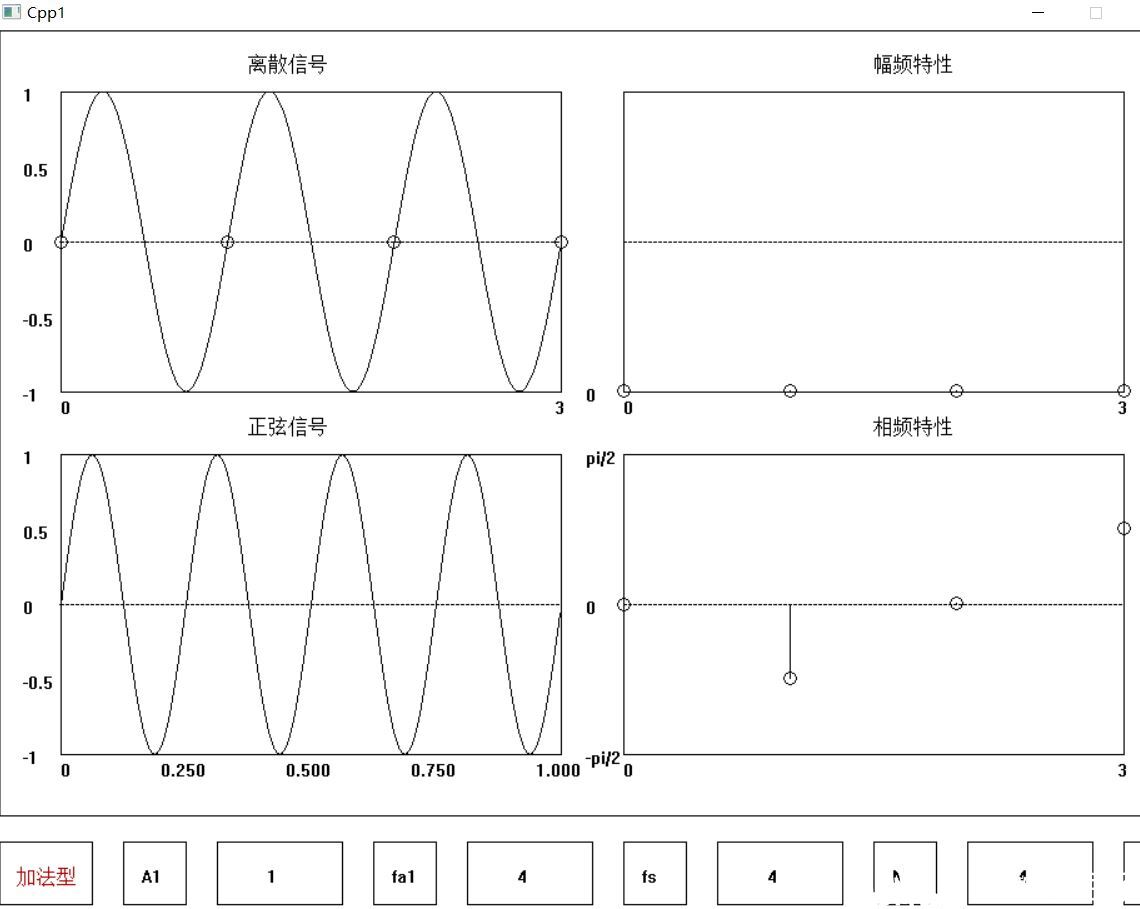上图是fs=fa，幅频图失真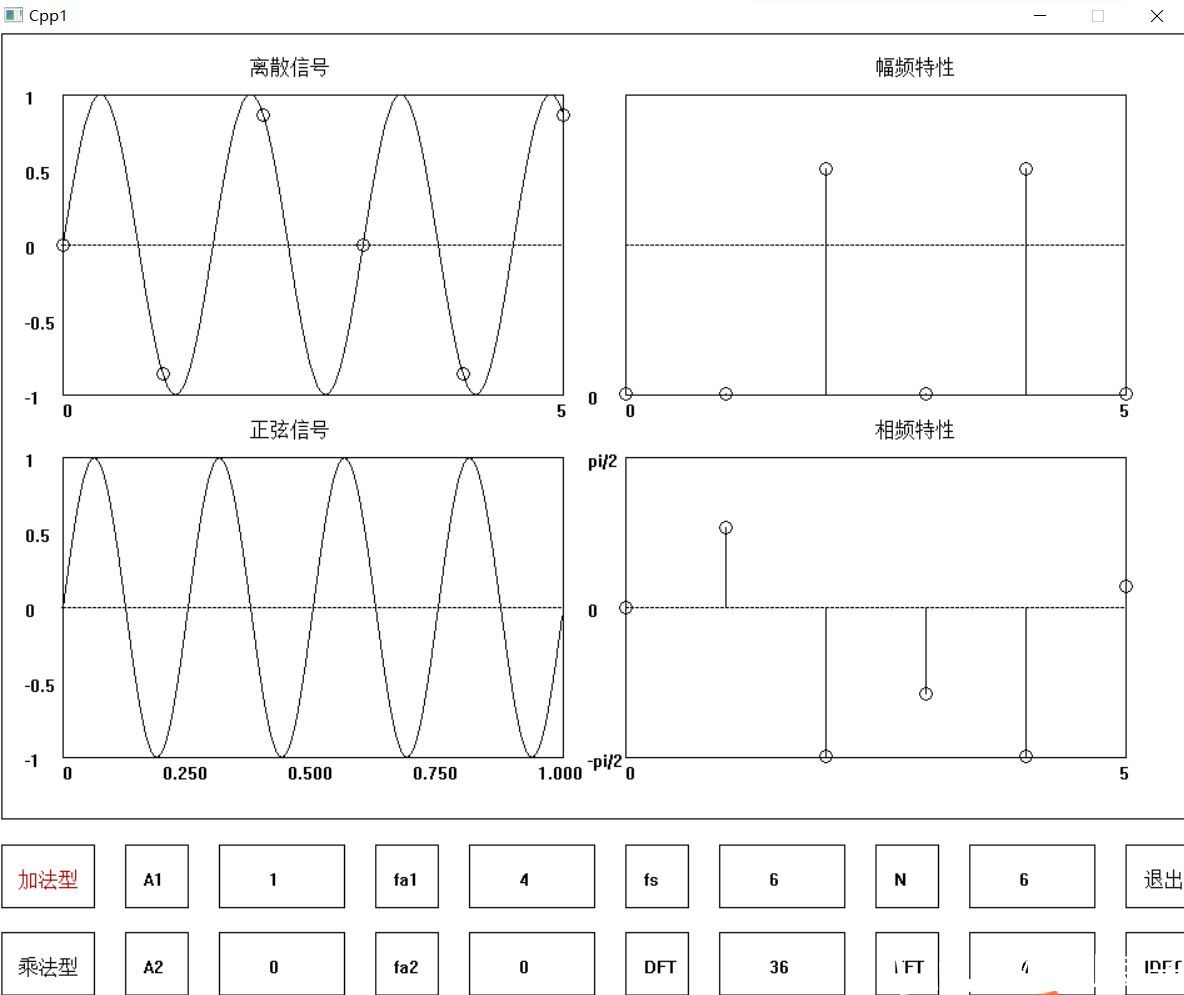上图是fs=1.5fa，DFT后，频谱图似乎显示是正常的上图是fs=1.5fa，IDFT后会发现恢复的信号失真了，频率降半了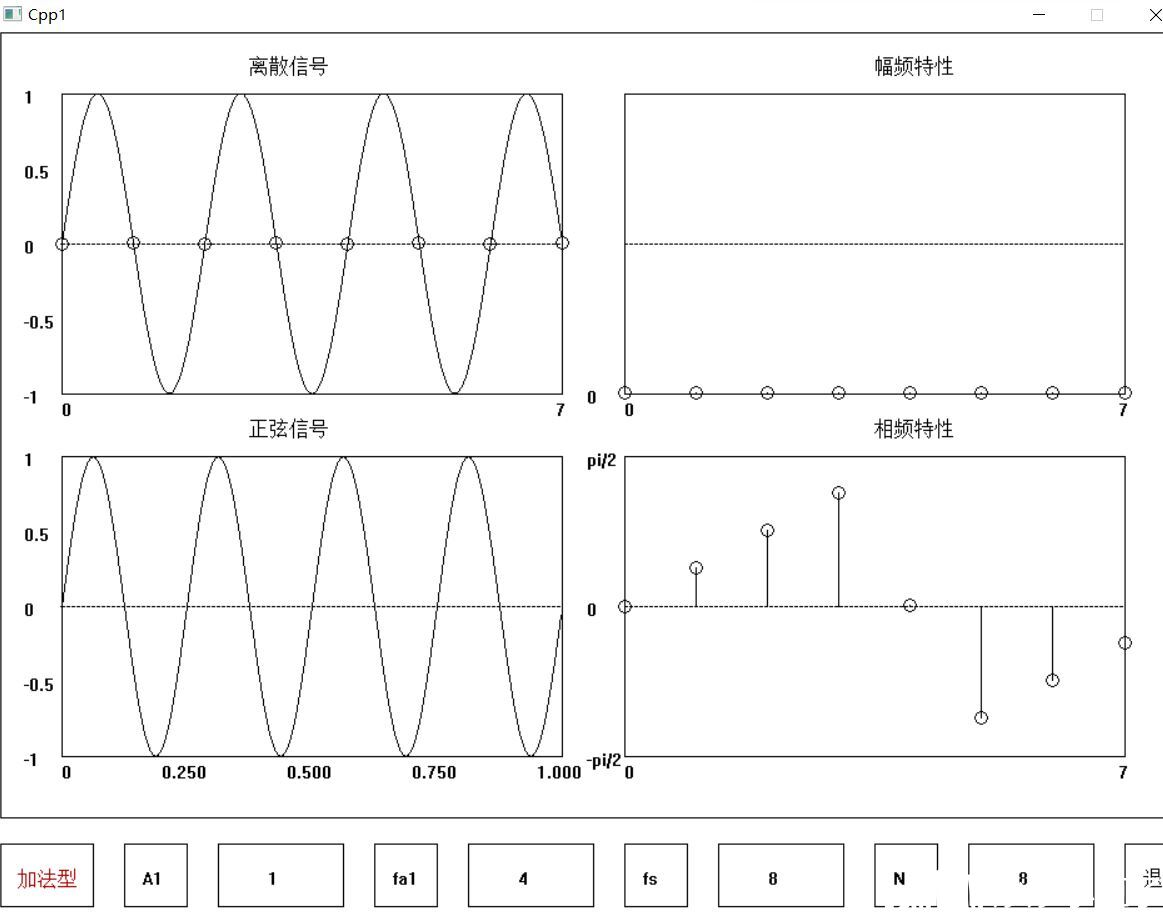上图是fs=2fa，幅频图失真7.  泄露(N不是fs/fa的整数倍)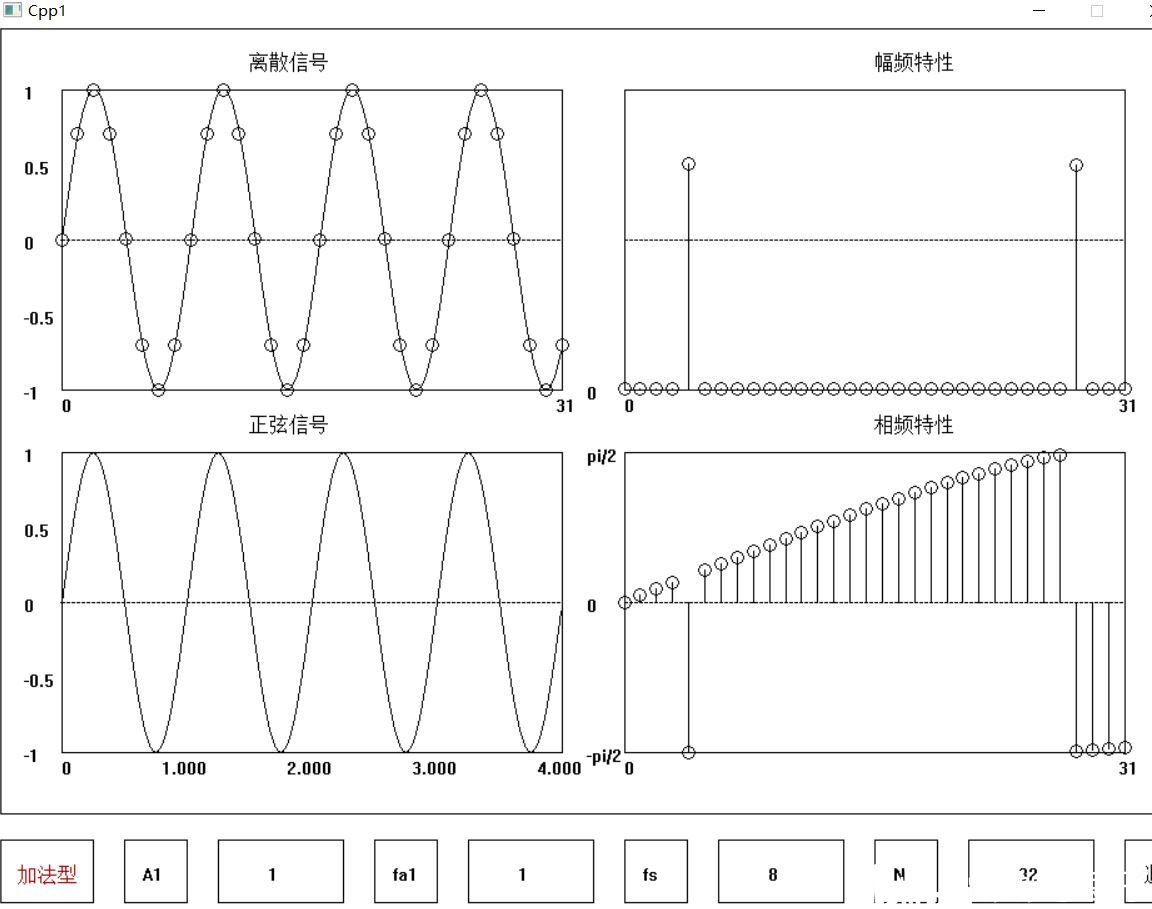上图是fs=8fa，N=4fs/fa，频谱图正常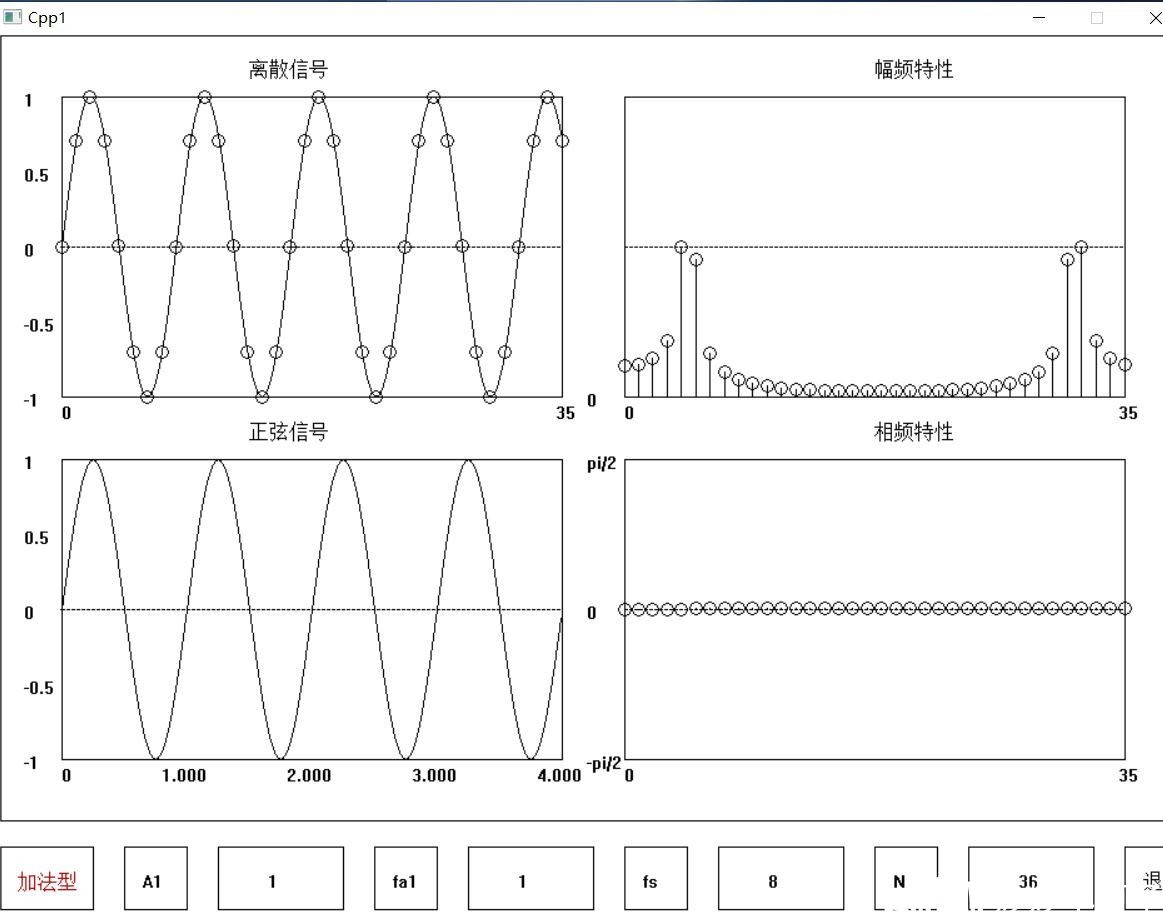上图是fs=8fa，N=4.5fs/fa，频谱图泄露8.  IDFT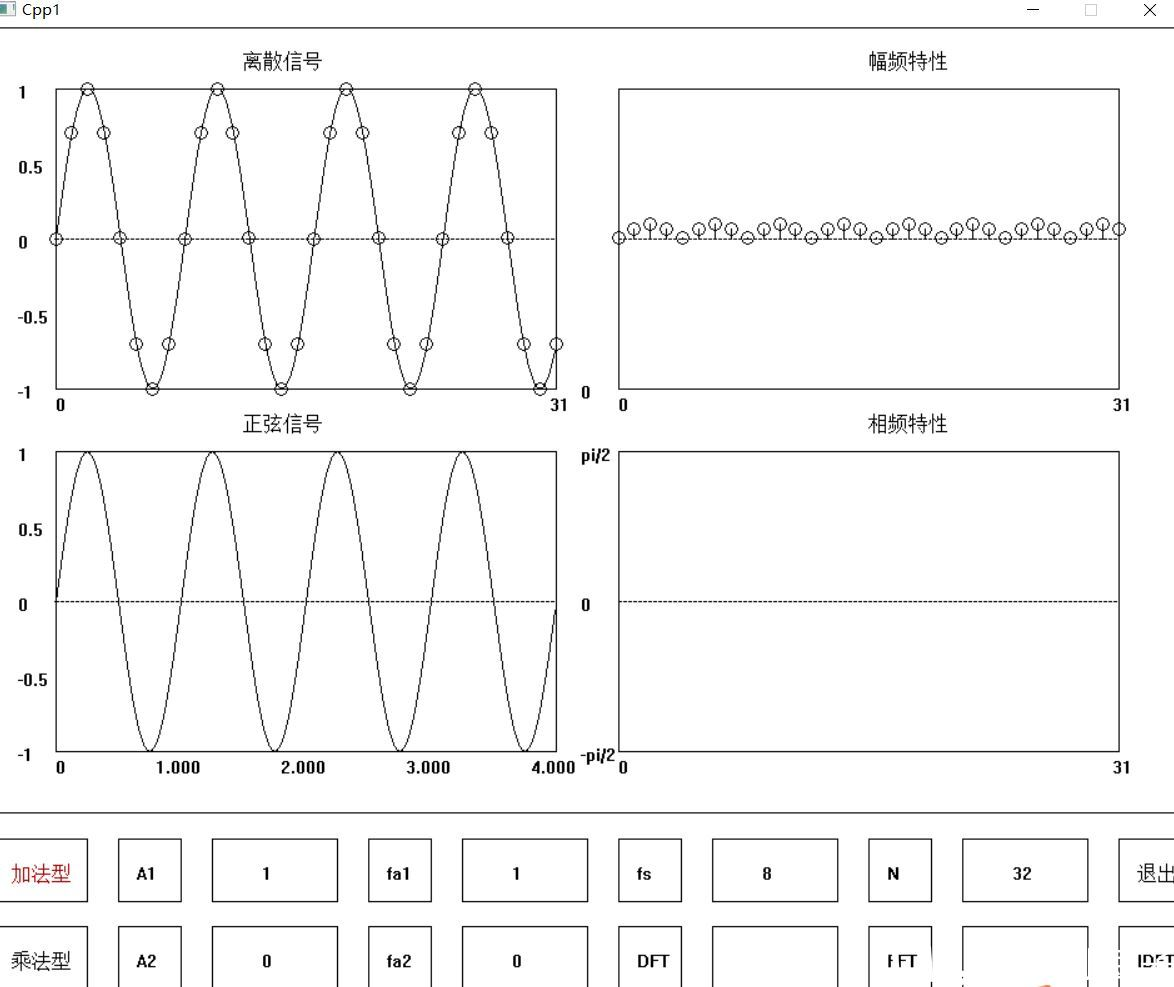上图是fs=8fa，N=4fs/fa，DFT执行得到X(n)后，IDFT的结果代码：#include #include #include #include #include #include #include #include #include #include #include #include #include using namespace std;HWND hOut;struct Node{double Re;double Im;};double A1,A2,fa1,fa2,fa,fs,N,pi,c1,c2;double xt;double xn;Node Xn;MOUSEMSG m;int exit1,exit2;int mod;double t1,t2;void IDFT(){int m,n;double I,J;for(m=0;m<=N-1;m++){xn[m]=0;I=0;J=0;for(n=0;n<=N-1;n++){I=I+double(Xn[n].Re)*cos(2.0*pi*double((n*m)/N))-double(Xn[n].Im)*sin(2.0*pi*double((n*m)/N));J=J+double(Xn[n].Im)*cos(2.0*pi*double((n*m)/N))+double(Xn[n].Re)*sin(2.0*pi*double((n*m)/N));}xn[m]=sqrt(I*I+J*J)/N;line(500+m*400.0/(N-1),170,500+m*400.0/(N-1),170-xn[m]);circle(500+m*400.0/(N-1),170-xn[m],5);}}void FFT(){int m,n;double I,J;char s;double I1,J1;sprintf(s, "%0.0f", t1);outtextxy(625-10, 720+20, s);t2=0;for(m=0;m<=N/2-1;m++){I=0;J=0;I1=0;J1=0;for(n=0;n<=N/2-1;n++){I=I+xn[2*n]*cos(4.0*pi*double(n*m)/N)/10;J=J-xn[2*n]*sin(4.0*pi*double(n*m)/N)/10;I1=I1+xn[2*n+1]*cos(2.0*pi*double((2*n+1)*m)/N)/10;J1=J1-xn[2*n+1]*sin(2.0*pi*double((2*n+1)*m)/N)/10;t2++;}Xn[m].Re=I+I1;Xn[m].Im=J+J1;Xn[int(m+N/2)].Re=I-I1;Xn[int(m+N/2)].Im=J-J1;line(500+double(m)*400.0/(N-1),290,500+double(m)*400.0/(N-1),290-sqrt(Xn[m].Re*Xn[m].Re+Xn[m].Im*Xn[m].Im));circle(500+double(m)*400.0/(N-1),290-sqrt(Xn[m].Re*Xn[m].Re+Xn[m].Im*Xn[m].Im),5);line(500+(m+N/2)*400.0/(N-1),290,500+(m+N/2)*400.0/(N-1),290-sqrt(Xn[int(m+N/2)].Re*Xn[int(m+N/2)].Re+Xn[int(m+N/2)].Im*Xn[int(m+N/2)].Im));circle(500+(m+N/2)*400.0/(N-1),290-sqrt(Xn[int(m+N/2)].Re*Xn[int(m+N/2)].Re+Xn[int(m+N/2)].Im*Xn[int(m+N/2)].Im),5);if(Xn[m].Re!=0){line(500+m*400.0/(N-1),460,500+m*400.0/(N-1),460-atan(Xn[m].Im/Xn[m].Re)/(pi/2)*120.0);circle(500+m*400.0/(N-1),460-atan(Xn[m].Im/Xn[m].Re)/(pi/2)*120.0,5);}if(Xn[int(m+N/2)].Re!=0){line(500+(m+N/2)*400.0/(N-1),460,500+(m+N/2)*400.0/(N-1),460-atan(Xn[int(m+N/2)].Im/Xn[int(m+N/2)].Re)/(pi/2)*120.0);circle(500+(m+N/2)*400.0/(N-1),460-atan(Xn[int(m+N/2)].Im/Xn[int(m+N/2)].Re)/(pi/2)*120.0,5);}}sprintf(s, "%0.0f", t2);outtextxy(825-10, 720+20, s);}void DFT(){int m,n;char s;sprintf(s, "%0.0f", t2);outtextxy(825-10, 720+20, s);t1=0;for(m=0;m<=N-1;m++){Xn[m].Re=0;Xn[m].Im=0;for(n=0;n<=N-1;n++){Xn[m].Re=Xn[m].Re+xn[n]*cos(2.0*pi*double(n*m)/N)*3/N;Xn[m].Im=Xn[m].Im-xn[n]*sin(2.0*pi*double(n*m)/N)*3/N;t1++;}line(500+double(m)*400.0/(N-1),290,500+double(m)*400.0/(N-1),290-sqrt(Xn[m].Re*Xn[m].Re+Xn[m].Im*Xn[m].Im));circle(500+double(m)*400.0/(N-1),290-sqrt(Xn[m].Re*Xn[m].Re+Xn[m].Im*Xn[m].Im),5);if(Xn[m].Re!=0){line(500+m*400.0/(N-1),460,500+m*400.0/(N-1),460-atan(Xn[m].Im/Xn[m].Re)/(pi/2)*120.0);circle(500+m*400.0/(N-1),460-atan(Xn[m].Im/Xn[m].Re)/(pi/2)*120.0,5);}}sprintf(s, "%0.0f", t1);outtextxy(625-10, 720+20, s);}void Sample(){int i,j,k,n,m;double I,J,K;j=460;n=170;for(i=0;i<=399;i++){if(mod==1){k=A1*(120.0/(A1+A2))*sin(2.0*pi*fa1*double(i)/100.0/fa)+A2*(120.0/(A1+A2))*sin(2.0*pi*fa2*double(i)/100.0/fa);xt[i]=A1*(120.0/(A1+A2))*sin(2.0*pi*fa1*double(N-1)*double(i)/400.0/fs)+A2*(120.0/(A1+A2))*sin(2.0*pi*fa2*double(N-1)*double(i)/400.0/fs);}else{k=120.0*sin(2.0*pi*fa1*double(i)/100.0/fa)*sin(2.0*pi*fa2*double(i)/100.0/fa);xt[i]=120.0*sin(2.0*pi*fa1*double(N-1)*double(i)/400.0/fs)*sin(2.0*pi*fa2*double(N-1)*double(i)/400.0/fs);}line(i+49,j,i+50,460-k);line(i+49,n,i+50,170-xt[i]);j=460-k;n=170-xt[i];}for(n=0;n<=N-1;n++){if(mod==1){xn[n]=A1*(120.0/(A1+A2))*sin(2.0*pi*fa1*double(n)/fs)+A2*(120.0/(A1+A2))*sin(2.0*pi*fa2*double(n)/fs);}else{xn[n]=120.0*sin(2.0*pi*fa1*double(n)/fs)*sin(2.0*pi*fa2*double(n)/fs);}circle(50+400*n/(N-1),170-xn[n],5);}}void draw(){char s;cleardevice();setlinecolor(BLACK);fillrectangle(1,1,949,629);fillrectangle(50,50,450,290);fillrectangle(500,50,900,290);fillrectangle(50,340,450,580);fillrectangle(500,340,900,580);setlinestyle(PS_DASH);line(50,170,450,170);line(500,170,900,170);line(50,460,450,460);line(500,460,900,460);setlinestyle(PS_SOLID);settextcolor(BLACK);outtextxy(20,45, _T("1"));//sprintf(s, "%01.0f", A1+A2);outtextxy(20,45, s);outtextxy(20,105, _T("0.5"));outtextxy(20,165, _T("0"));outtextxy(20,225, _T("-0.5"));outtextxy(20,285, _T("-1"));outtextxy(20,335, _T("1"));outtextxy(20,395, _T("0.5"));outtextxy(20,455, _T("0"));outtextxy(20,515, _T("-0.5"));outtextxy(20,575, _T("-1"));outtextxy(470,290-5, _T("0"));outtextxy(470,340-5, _T("pi/2"));outtextxy(470,460-5, _T("0"));outtextxy(470,580-5, _T("-pi/2"));outtextxy(50,290+5, _T("0"));outtextxy(50,580+5, _T("0"));outtextxy(500,290+5, _T("0"));outtextxy(500,580+5, _T("0"));outtextxy(200,20, _T("离散信号"));outtextxy(200,310, _T("正弦信号"));outtextxy(700,20, _T("幅频特性"));outtextxy(700,310, _T("相频特性"));sprintf(s, "%01.0f", N-1);outtextxy(450-5, 290+5, s);outtextxy(900-5, 290+5, s);outtextxy(900-5, 580+5, s);if(fa1==0&&fa2==0){fa=1;}else if(fa1==0){fa=fa2;}else if(fa2==0){fa=fa1;}else {fa=(fa1<=fa2?fa1:fa2);}sprintf(s, "%04.3f", 1/fa);outtextxy(150-20, 580+5, s);sprintf(s, "%04.3f", 2/fa);outtextxy(250-20, 580+5, s);sprintf(s, "%04.3f", 3/fa);outtextxy(350-20, 580+5, s);sprintf(s, "%04.3f", 4/fa);outtextxy(450-20, 580+5, s);fillrectangle(1,650,50+25,700);fillrectangle(100,650,150,700);fillrectangle(200-25,650,250+25,700);//fillrectangle(250,650,300,700);fillrectangle(300,650,350,700);fillrectangle(400-25,650,450+25,700);fillrectangle(500,650,550,700);fillrectangle(600-25,650,650+25,700);fillrectangle(700,650,750,700);fillrectangle(800-25,650,850+25,700);fillrectangle(900,650,949,700);fillrectangle(1,720,50+25,770);fillrectangle(100,720,150,770);fillrectangle(200-25,720,250+25,770);fillrectangle(300,720,350,770);fillrectangle(400-25,720,450+25,770);fillrectangle(500,720,550,770);fillrectangle(600-25,720,650+25,770);fillrectangle(700,720,750,770);fillrectangle(800-25,720,850+25,770);fillrectangle(900,720,949,770);if(mod==1)settextcolor(RED);outtextxy(25-10,650+20, _T("加法型"));settextcolor(BLACK);outtextxy(125-10,650+20, _T("A1"));sprintf(s, "%0.0f", A1);outtextxy(225-10, 650+20, s);outtextxy(325-10,650+20, _T("fa1"));sprintf(s, "%0.0f", fa1);outtextxy(425-10, 650+20, s);outtextxy(525-10,650+20, _T("fs"));sprintf(s, "%0.0f", fs);outtextxy(625-10, 650+20, s);outtextxy(725-10,650+20, _T("N"));sprintf(s, "%0.0f", N);outtextxy(825-10, 650+20, s);outtextxy(925-10,650+20, _T("退出"));if(mod==2)settextcolor(RED);outtextxy(25-10,720+20, _T("乘法型"));settextcolor(BLACK);outtextxy(125-10,720+20, _T("A2"));sprintf(s, "%0.0f", A2);outtextxy(225-10, 720+20, s);outtextxy(325-10,720+20, _T("fa2"));sprintf(s, "%0.0f", fa2);outtextxy(425-10, 720+20, s);outtextxy(525-10,720+20, _T("DFT"));//sprintf(s, "%0.0f", t1);outtextxy(625-10, 720+20, s);outtextxy(725-10,720+20, _T("FFT"));//sprintf(s, "%0.0f", t2);outtextxy(825-10, 720+20, s);outtextxy(925-10,720+20, _T("IDFT"));Sample();}void initialization(){hOut=initgraph(950, 772);//,SHOWCONSOLE);setbkcolor(WHITE);cleardevice();A1=1;fa1=1;A2=0;fa2=0;fa=1;fs=8;N=32;t1=0;t2=0;exit1=0;exit2=0;mod=1;pi=3.141592653;}void move(){char ss;int i;draw();while(exit2==0){if(MouseHit()){m=GetMouseMsg();if(m.uMsg==WM_LBUTTONDOWN){if(m.x>=175&&m.x<=275&&m.y>=630&&m.y<=680){InputBox(ss, 10, "设置A1");sscanf(ss, "%d", &i);if(i>=-400&&i<=400){A1=i;}else {MessageBox(hOut,"输入错误,不在范围内","来自小豆子的提醒",MB_OK);}draw();}else if(m.x>=375&&m.x<=475&&m.y>=630&&m.y<=680){InputBox(ss, 10, "设置fa1");sscanf(ss, "%d", &i);if(i>=-400&&i<=400){fa1=i;}else {MessageBox(hOut,"输入错误,不在范围内","来自小豆子的提醒",MB_OK);}draw();}else if(m.x>=575&&m.x<=675&&m.y>=630&&m.y<=680){InputBox(ss, 10, "设置fs");sscanf(ss, "%d", &i);if(i>=-400&&i<=400){fs=i;}else {MessageBox(hOut,"输入错误,不在范围内","来自小豆子的提醒",MB_OK);}draw();}else if(m.x>=775&&m.x<=875&&m.y>=630&&m.y<=680){InputBox(ss, 10, "设置N");sscanf(ss, "%d", &i);if(i>=-400&&i<=400){N=i;}else {MessageBox(hOut,"输入错误,不在范围内","来自小豆子的提醒",MB_OK);}draw();}else if(m.x>=175&&m.x<=275&&m.y>=720&&m.y<=770){InputBox(ss, 10, "设置A2");sscanf(ss, "%d", &i);if(i>=-400&&i<=400){A2=i;}else {MessageBox(hOut,"输入错误,不在范围内","来自小豆子的提醒",MB_OK);}draw();}else if(m.x>=375&&m.x<=475&&m.y>=720&&m.y<=770){InputBox(ss, 10, "设置fa2");sscanf(ss, "%d", &i);if(i>=-400&&i<=400){fa2=i;}else {MessageBox(hOut,"输入错误,不在范围内","来自小豆子的提醒",MB_OK);}draw();}else if(m.x>=500&&m.x<=550&&m.y>=720&&m.y<=770){draw();DFT();}else if(m.x>=700&&m.x<=750&&m.y>=720&&m.y<=770){draw();FFT();}else if(m.x>=900&&m.x<=950&&m.y>=720&&m.y<=770){draw();IDFT();}else if(m.x>=1&&m.x<=75&&m.y>=630&&m.y<=680){mod=1;A1=1;A2=0;fa1=1;fa2=0;draw();}else if(m.x>=1&&m.x<=75&&m.y>=720&&m.y<=770){mod=2;A1=1;A2=1;fa1=1;fa2=1;draw();}}}}}void carry(){initialization();while(exit1==0){move();}closegraph();}int main(){carry();}演示视频：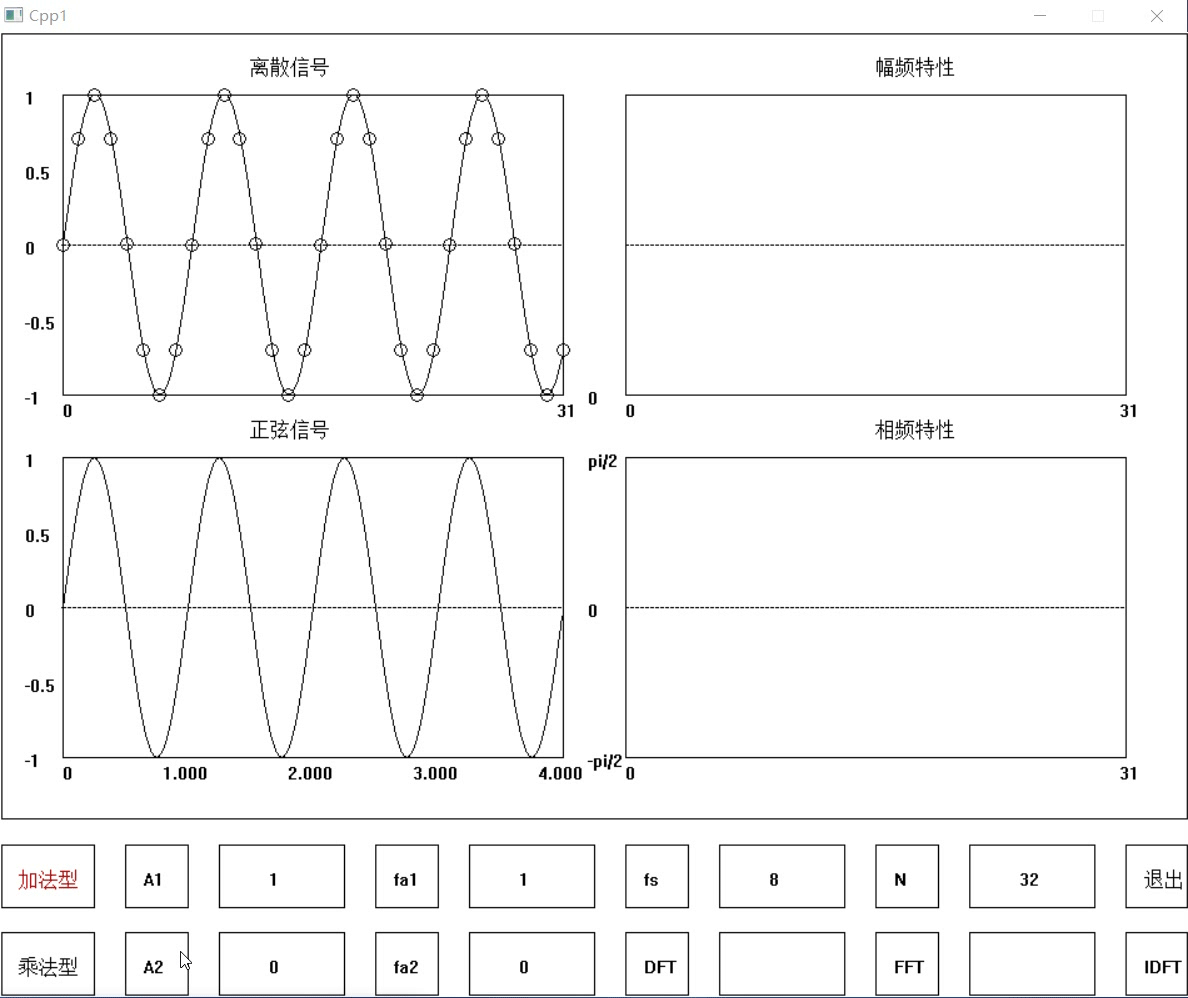展开全文• 角频率或频率为横轴，振幅和相位为纵轴，画一个坐标系，表示这个正弦信号 在新坐标系（角频率或频率为横轴，，振幅和相位为纵轴）中，以两条线（甚至两个点就够了），表示了时域波形如图2.1所示信号，或者说...
基本概念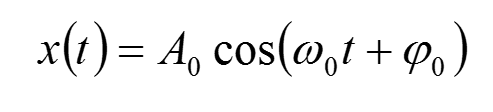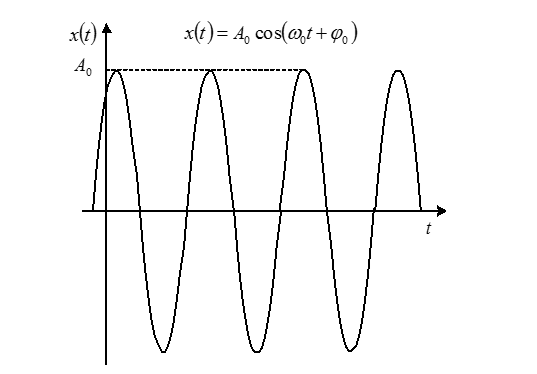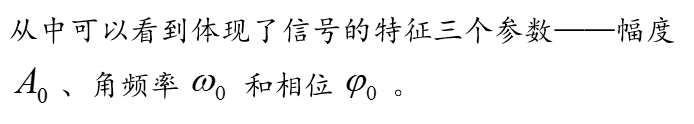欧拉公式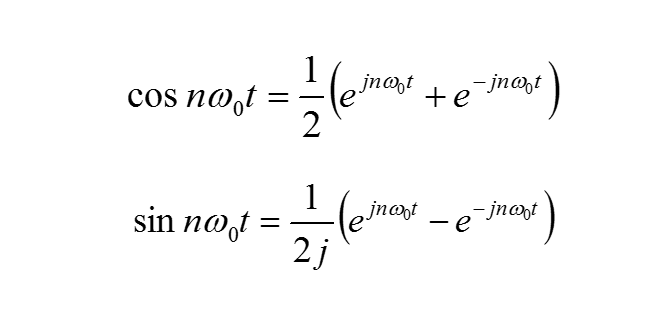根据欧拉公式正余弦信号还可以被指数形式所表示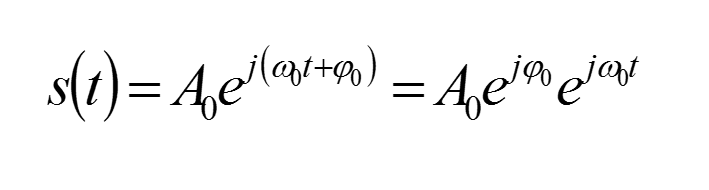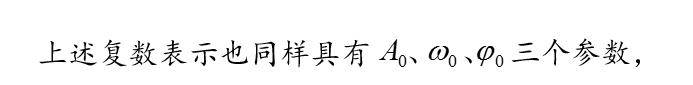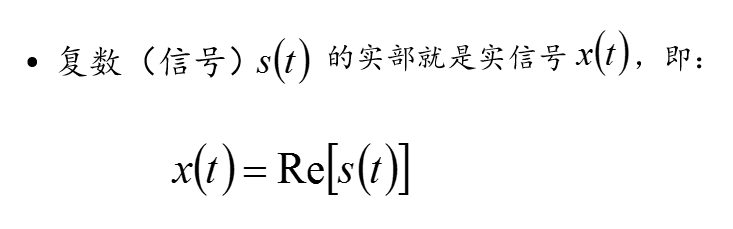角频率或频率为横轴，振幅和相位为纵轴，画一个坐标系，表示这个正弦信号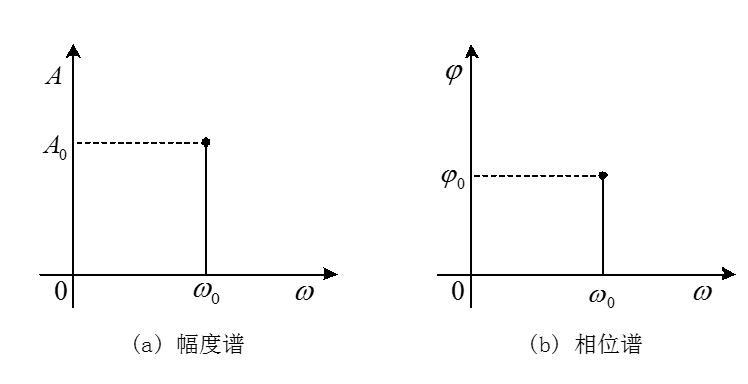在新的坐标系（角频率或频率为横轴，，振幅和相位为纵轴）中，以两条线（甚至两个点就够了），表示了时域波形如图2.1所示的信号，或者说，表示了信号所有的特征信息（频率、幅度和相位）。这种表示法被称为频域表示，表示的结果叫做“频谱”，对应于振幅或者相位分别为幅度谱和相位谱。

上述正弦信号只有单一频率，因此其频谱只包含一根“线”（谱线），人们常称其为“单色”信号。

傅里叶级数

公式推导，百度或者数学课本。

任何周期信号都能够由不同谐波的正弦波叠加而成，这由傅里叶发现，因此称之为傅里叶级数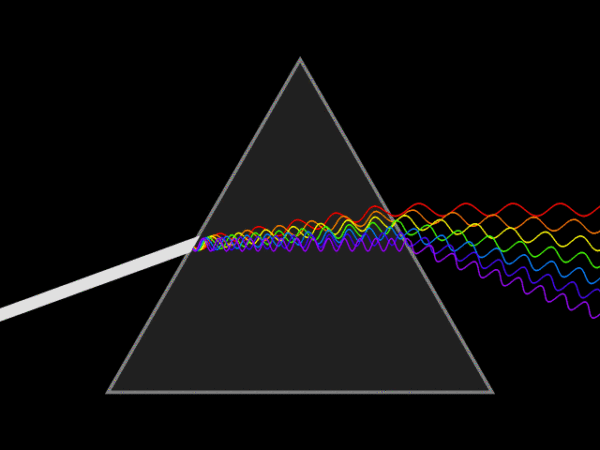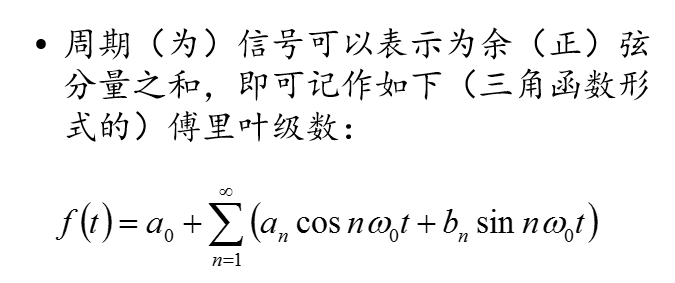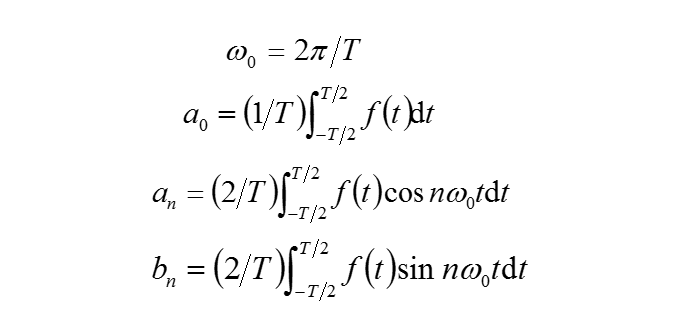可以通过积化和差，把sin和cos化为一个cos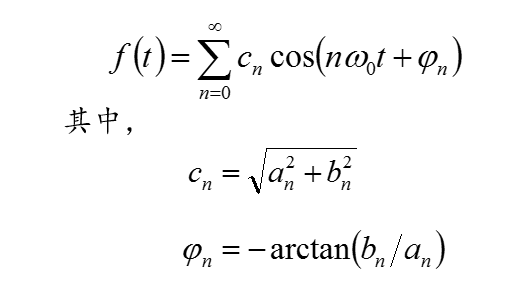如果我把一个信号的所有正弦分量的频率算出来画出来，那么就如下图 ，也叫频谱图

频谱是频率谱密度的简称，是频率的分布曲线。复杂振荡分解为振幅不同和频率不同的谐振荡，这些谐振荡的幅值按频率排列的图形叫做频谱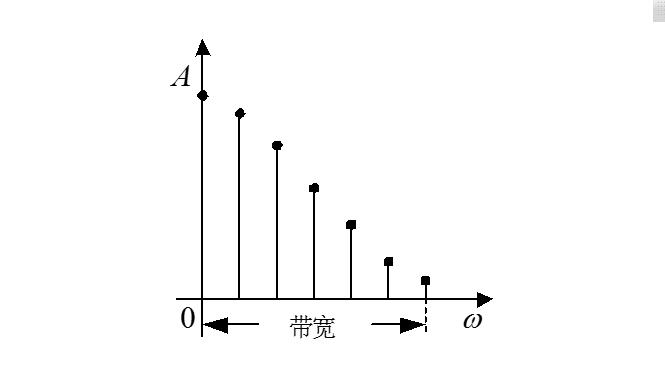考察某个信号的所有正弦分量，这些正弦分量覆盖的频率范围，被形象地叫做“频带”。这个范围的大小，就是“带宽”——即频带宽度，如图所示。带宽是衡量信号特性的一个重要指标。

极端情况下，相邻谱线足够接近时，频谱就可表示成连续的曲线了，原来分立的谱线于是简化为曲线中的一个个点 。

根据欧拉公式也可以用指数来表示一个周期信号

欧拉公式因此傅里叶级数还可以表示成以下指数形式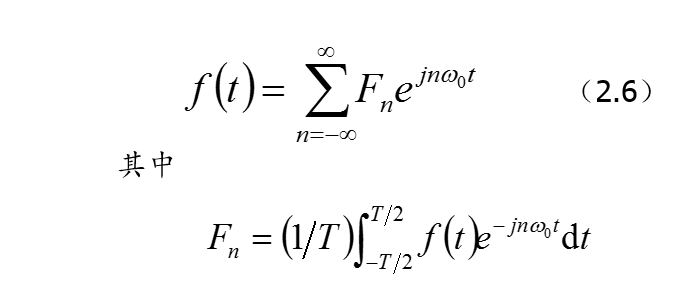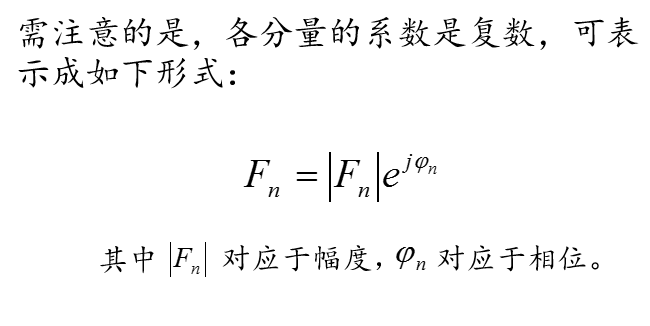如果我把一个信号的所有指数分量的频率算出来画出来，那么就如下图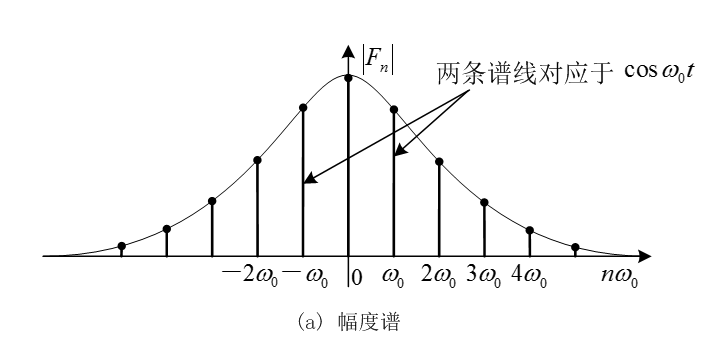可以看到，复频谱除正频率分量外，还包括负频率分量。负频率的出现是数学运算（欧拉公式）的结果，并无物理意义。

总结：

上述正弦信号只有单一频率，因此其频谱只包含一根“线”（谱线），人们常称其为“单色”信号。而在大多数应用场合中，信号是由若干不同频率的单色信号叠加而成的，称为“复合”信号。从频域角度看，复合信号的频谱包含若干条甚至无数条谱线。

MATLAB编程实现：y=10+A*sin(2*pi*10*x)+5*sin(2*pi*20*x);

画图用点*不要用-，不然以为没有采样点。时间-幅度图，频率-幅度图


fs=200;%采样频率  是最高频率的两倍以上
t=1/fs;%采样间隔时长
T=2;%采样窗口长度
A=10;%幅度
N=T*fs% 采样点数 T/t
x=[0:(1/fs):T];%周期
len=length(x)-1;%x=N
y=10+A*sin(2*pi*10*x)+5*sin(2*pi*20*x);
subplot(3,1,1);
plot(x,y,'r*');
xlabel('时间/s');
ylabel('幅度');
subplot(3,1,2);
[f,y]=get_fft(y,fs,N);
plot(f,y);
xlabel('f/Hz');
ylabel('幅度');

function [f, spectrum ] = get_fft(s,Fs,L)
%GAN_FFT 此处显示有关此函数的摘要
%   此处显示详细说明
y=fft(s);
p2=abs(y/L);
p1=p2(1:L/2+1);
p1(2:end-1)=2*p1(2:end-1);
f = Fs*(0:(L/2))/L;
spectrum=p1;

end
%plot(f,spectrum);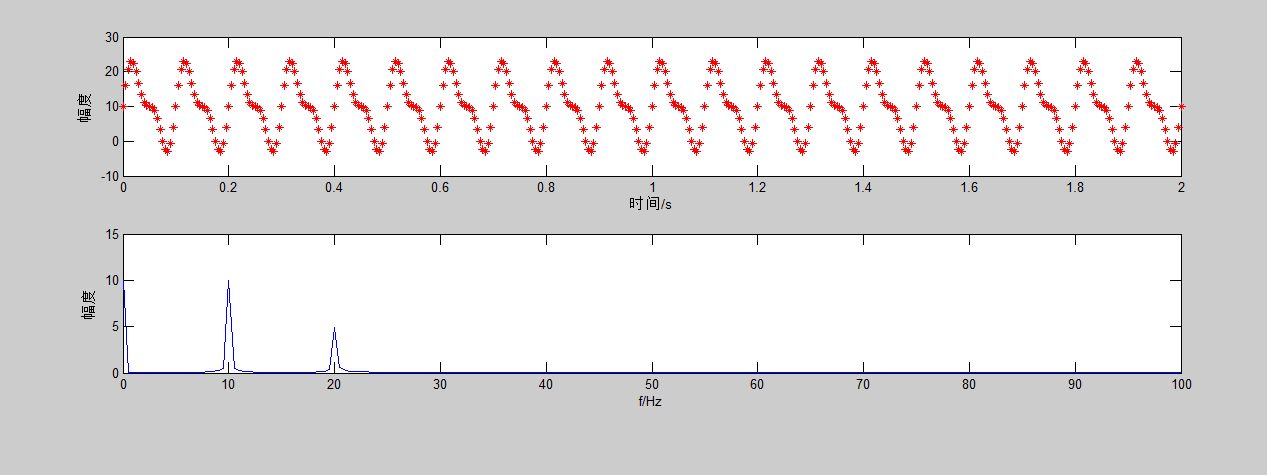以周期矩形信号为例，从傅里叶级数到傅里叶变换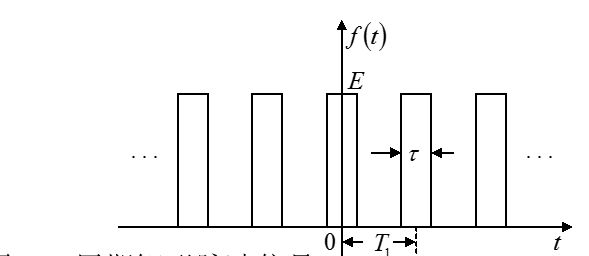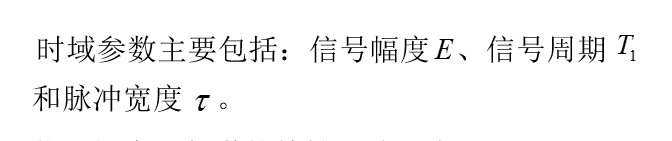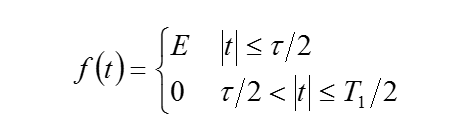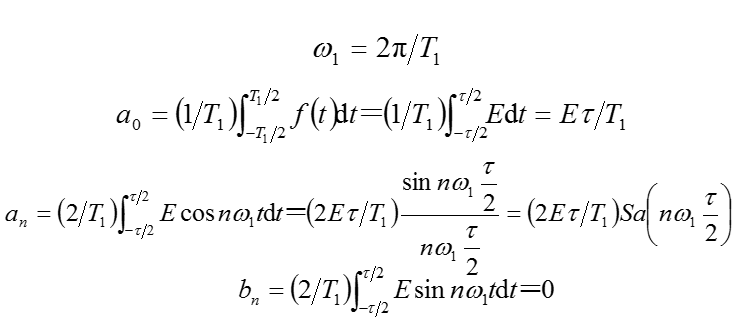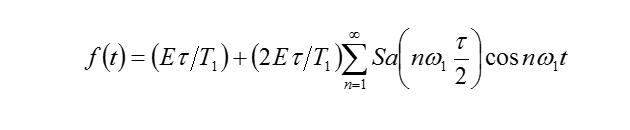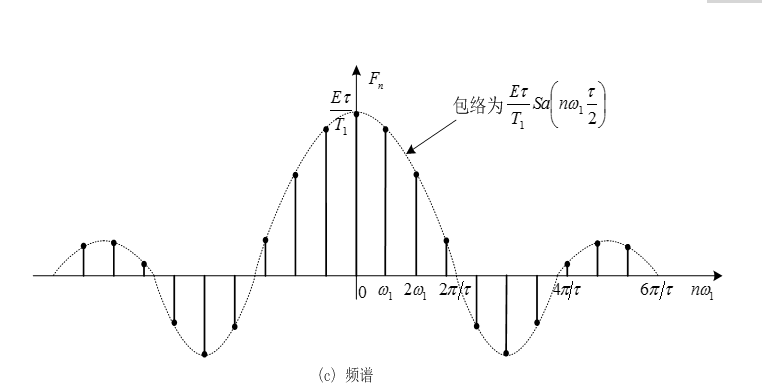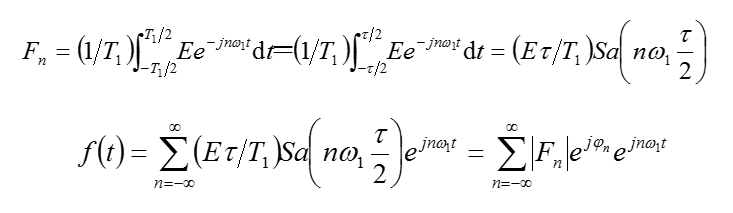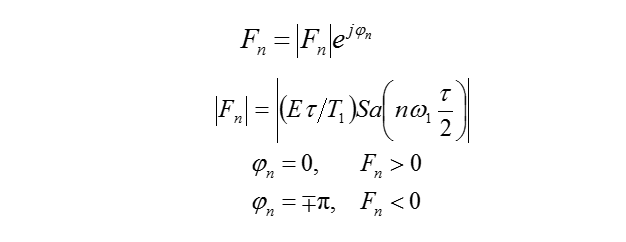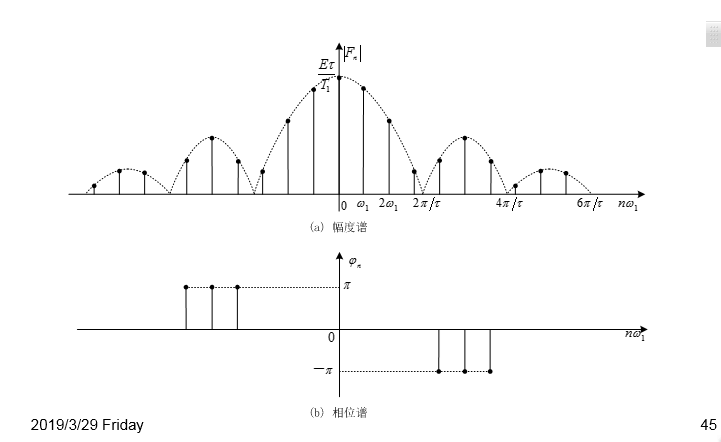突然发现好多数学式子啊，知道它是很多正弦信号组成的，那我们来大概看看，小的正弦信号怎么组成了这个周期矩形信号有没有发现前面的正弦信号，矩形脉冲信号，都是周期信号。那么非周期信号怎么办呢？

傅里叶认为，既然周期信号可以用正弦信号来表示，那么非周期信号也可以用正弦信号来逼近。原因是非周期信号可以看成是周期无限大

的周期信号；事实证明，傅里叶的想法是对的，于是才有了大名鼎鼎的傅里叶变换。

再回顾一下傅里叶级数的三角函数形式由于谱间隔为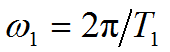另外谱的幅度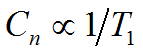所以当周期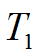无限增大时，谱线间隔会变密，而谱的幅度会减小。

极端情况下，若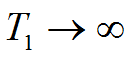，周期函数转换为非周期函数，这时离散频谱将成为连续频谱，分量幅值趋于无穷小。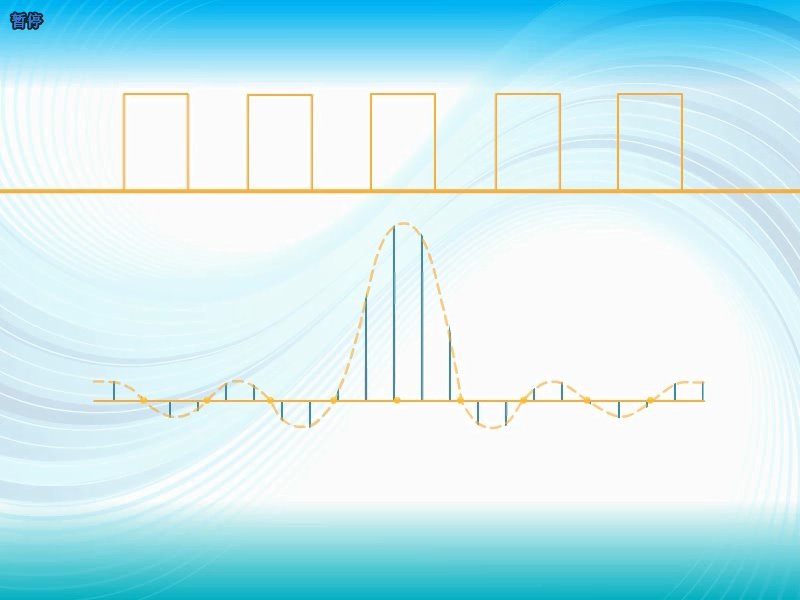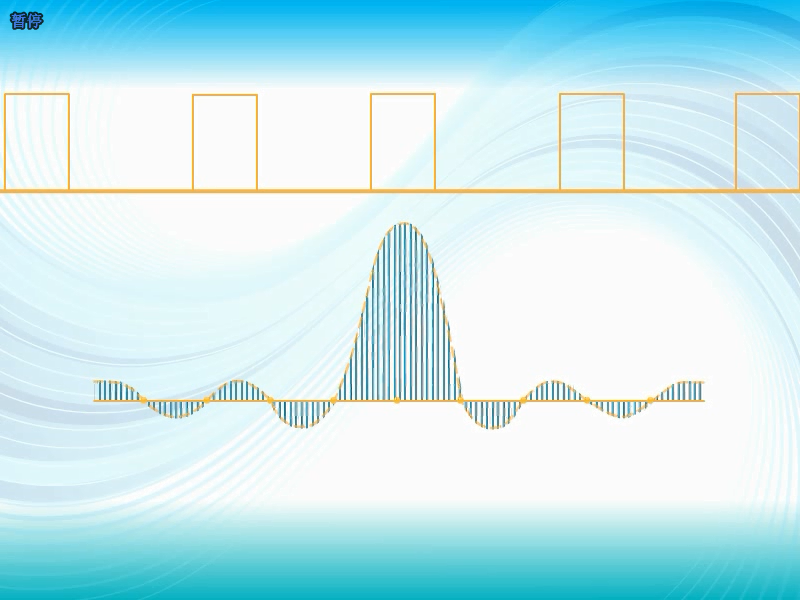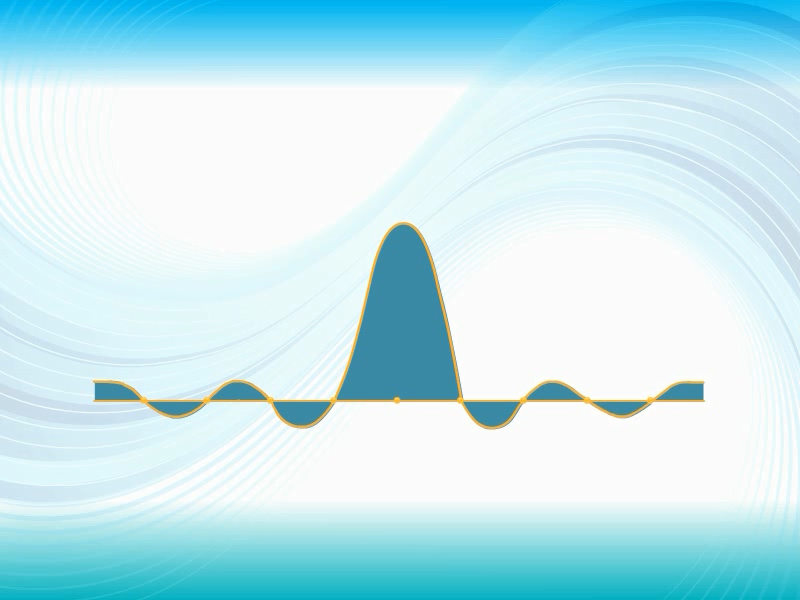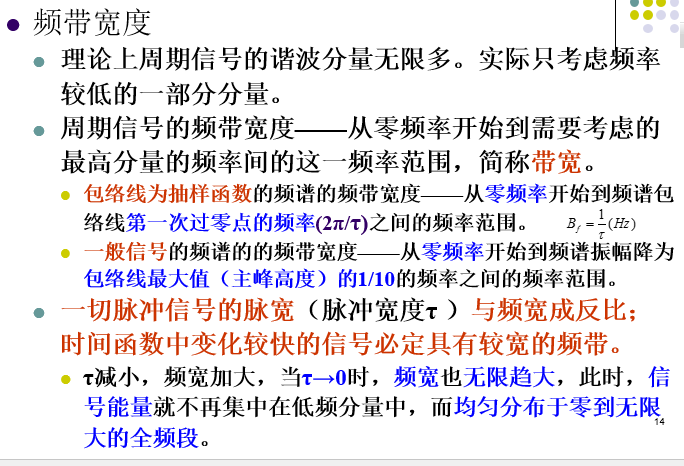由于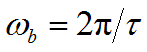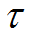增大时，带宽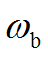减小减小时，带宽增大

这反映了一个普遍的规律：时域上压缩，减小，频域上展宽，增大

考虑一个极端情况，若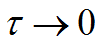，即矩形脉冲变成冲击函数

，则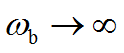，频谱的高阶谐波分量不衰减，成为所谓的白色谱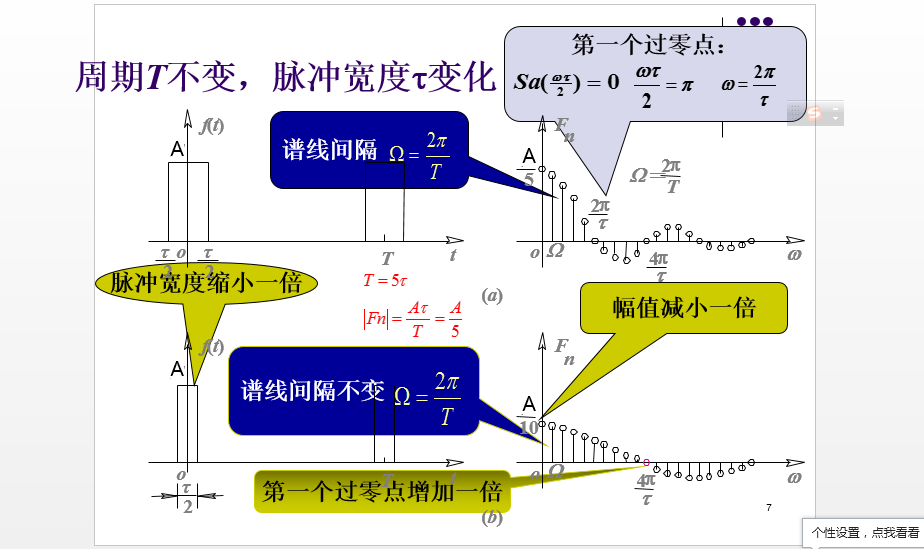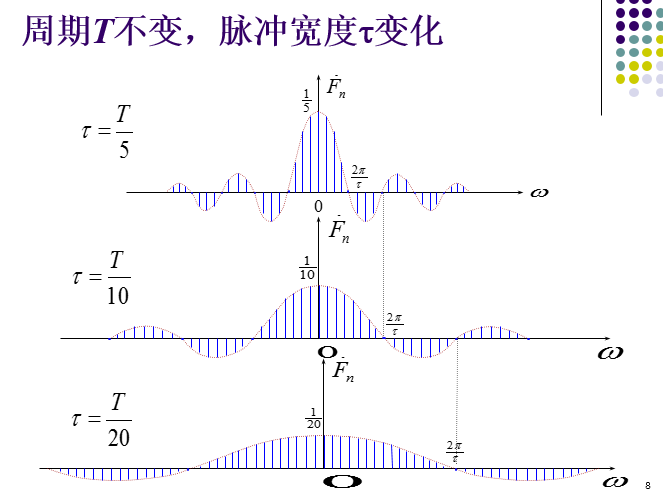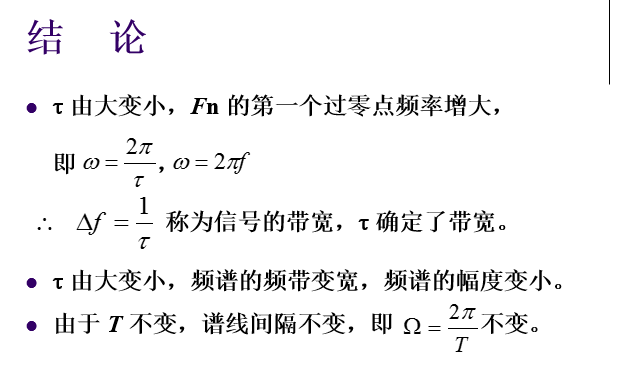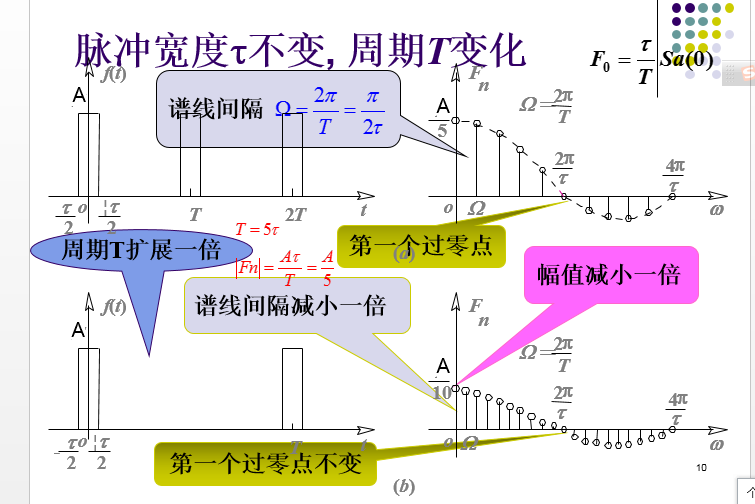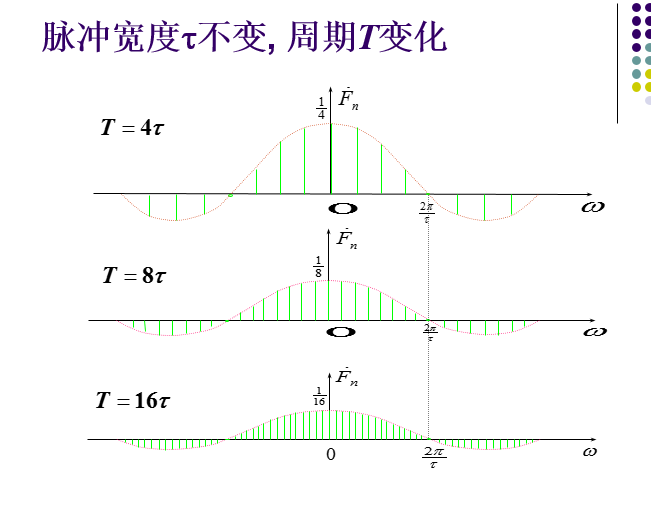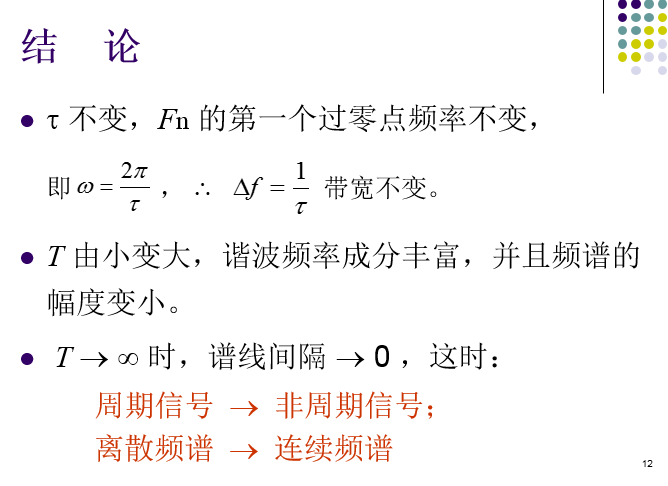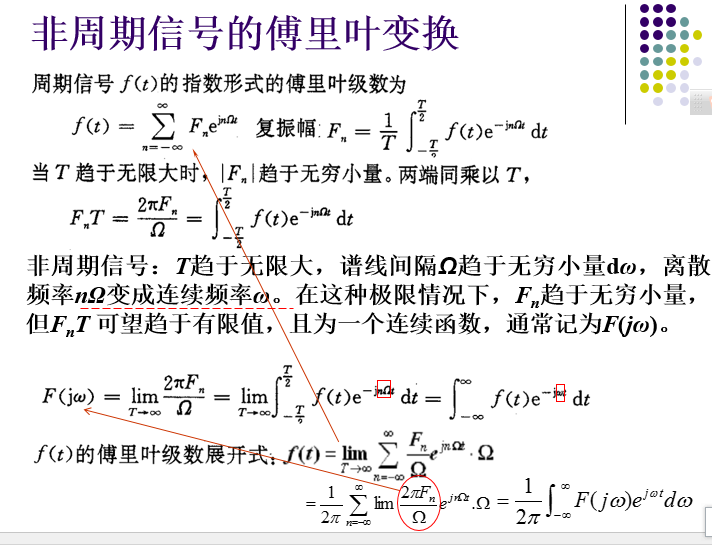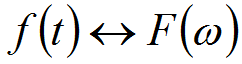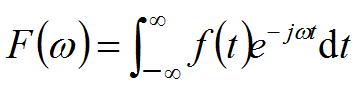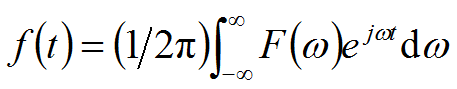展开全文• 正弦信号做快速傅里叶变换 FFT，即为快速傅氏变换，是离散傅氏变换快速算法，它是根据离散傅氏变换奇、偶、虚、实等特性，对离散傅立叶变换算法进行改进获得。它对傅氏变换理论并没有新发现，但是对于...
正弦信号做快速傅里叶变换

FFT，即为快速傅氏变换，是离散傅氏变换的快速算法，它是根据离散傅氏变换的奇、偶、虚、实等特性，对离散傅立叶变换的算法进行改进获得的。它对傅氏变换的理论并没有新的发现，但是对于在计算机系统或者说数字系统中应用离散傅立叶变换，可以说是进了一大步。

import matplotlib.pyplot as plt
import numpy as np
t = np.arange(256)
sp = np.fft.fft(np.sin(t))
freq = np.fft.fftfreq(t.shape[-1])
plt.plot(freq.tolist(), sp.real.tolist(), freq.tolist(), sp.imag.tolist())
plt.show()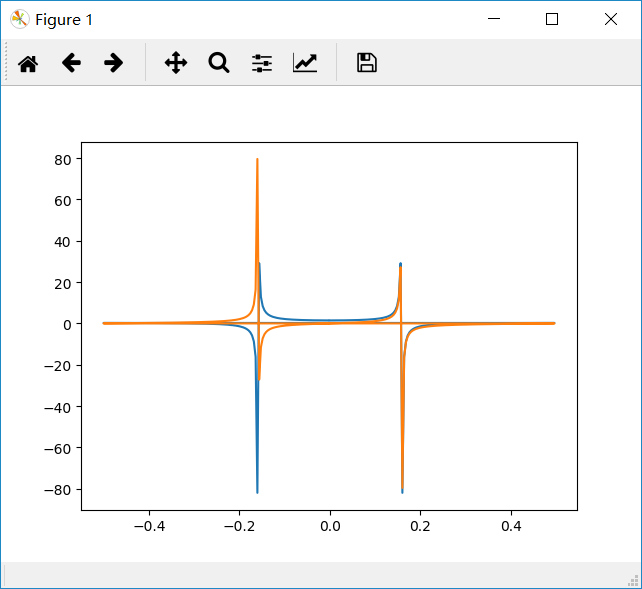展开全文python fft
• 在MATLAB中想要画一个sin函数是很容易。...应该借鉴数字信号处理里抽样思想：想生成函数x(t) = a*sin(2*pi*f0*t+ψ)，就应该考虑到抽样定理：以等时间间隔进行抽样，即x[n] = x[n*Ts] 其中Ts = ...
在MATLAB中想要画一个sin函数是很容易的。比如:首先定义t = 0:0.01:10,然后画出y = sin(2*pi*t)就可以了，最多再加相角啊之类的参数。但如果在C语言中应该如何自己制造一个sin函数呢？应该借鉴数字信号处理里的抽样思想：想生成函数x(t) = a*sin(2*pi*f0*t+ψ)，就应该考虑到抽样定理：以等时间间隔进行抽样，即x[n] = x[n*Ts] 其中Ts = 1/fs(fs是抽样频率)。这时候想生成的函数x(t)就变成了x(t) = x(n*Ts) = x(n) = a*sin(2*pi*f0*n/fs+ψ) = a*sin(w*n+ψ)，其中w是数字角频率，这就将模拟时间信号转化成了数字信号。举个例子，想生成函数y = sin(20*pi*t),用matlab直接生产的图形如下所示(取t=0:0.001：1)matlab程序为t = 0:0.001:1;y = sin(20*pi*t);plot(t,y);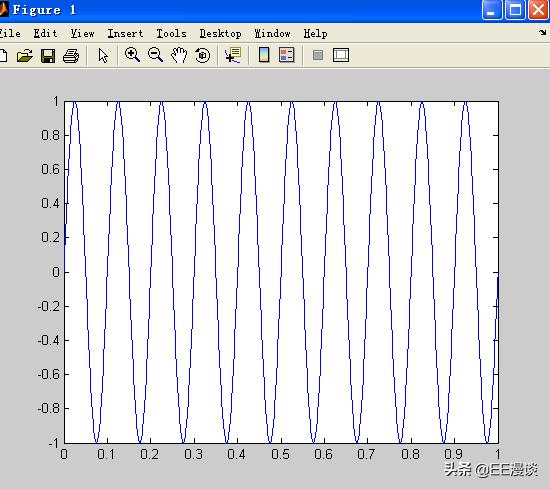如果想用C语言来实现怎么办呢？从原函数y = sin(20*pi*t)可知：f0 = 10,设fs = 40，n = 0:10(这里的n都是整数)来看看，此时x(n) = sin(2*pi*f0*n/fs)，matlab程序为clear all;f0=10;fs=40;N=10;n=0:N-1;y=sin(2*pi*n*f0/fs);画出来的图形如下图：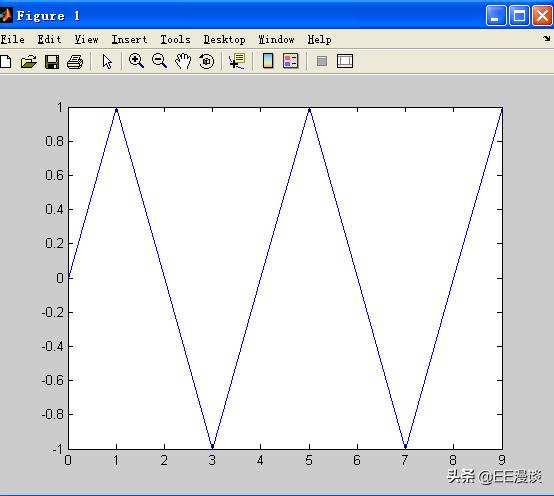现在函数式为x[n] = sin(n*pi/2),周期是4为什么看着不像以前的正弦函数了？因为这是对原函数抽样后得到的，而且它的横坐标已经变成了n而不是t。为了让抽样后的x[n]看起来更像x[t]那么方法只有一个，就是增加抽样率fs。当抽样率为80时x[n] = sin(n*pi/4),f0=10;fs=80;N=10;n=0:N-1;y=sin(2*pi*n*f0/fs);plot(n,y);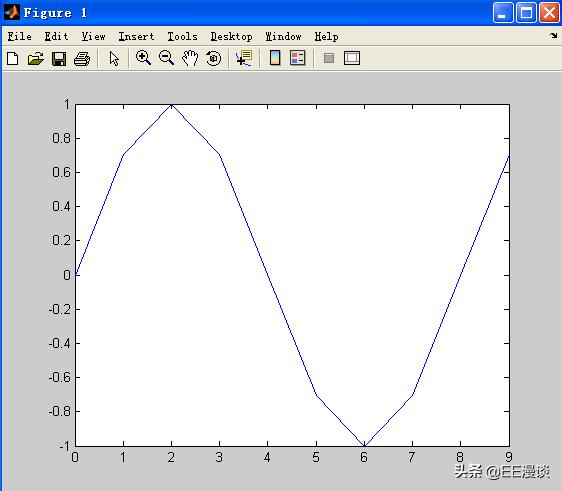看着更像x(t)了！这是因为抽样点更密了。所以产生x(t)=a*sin(2*pi*f0*t+ψ)的正弦波步骤：对x(t)进行以fs为抽样频率的抽样后得到x(n)=a*sin(2*pi*f0*n/fs+ψ)=a*sin(w*n+ψ)画的时候指明a，f0，fs，ψ就可以对任意sin函数进行画图那么，如何知道现在画出来的x(n)就是以前的x(t)呢？以前是T = 0.1S,现在变成了T = 8，在这种情况下如何换算呢？就有FFT了！下一篇文章我们再继续。
展开全文• Table of Contents 1.源代码 2.编译运行 CMakeLists.txt 编译 运行 致谢 1.源代码 #include <complex.h> #include <fftw3.h> #include <stdio.h> #include <...#...
• 通过傅里叶级数展开，将周期信号分解为一系列“加权”的正弦信号的叠加，对傅里叶级数展开各个频率分量的系数CnC_nCn​，通过将周期信号的周期趋近无穷大，进而得到了非周期信号的傅里叶变换F(jω)F(j\omega)F(jω)...
• 简单来说，傅里叶变换是将输入的信号分解成指定样式构造块。例如，首先通过叠加具有不同频率两个或更多个正弦函数而生成信号f(x)，之后，仅查看f(x)图像缺无法了解使用哪种或多少原始函数来生成f(x)。这就是傅...
• matlab实现两个正弦信号的混频并进行傅里叶变换（学会从频域去观察信号的特性）理论知识matlab代码信号分析 理论知识 以前也学过傅里叶变换的知识，但是一直没有理解进行傅里叶变换之后有什么作用，这次通过对两路...matlab fft
• 之前，我们已经了解到了周期信号可以分解为许多正弦信号（或者说，复指数信号）。只要给一个具体x(t)周期信号在一个周期上时域表示，我们就能求出来它在频域上表示。 但是问题又来了：如果信号是非周期呢...
• 7 时域、周期性、连续信号的傅里叶级数 7.1周期性、连续信号的傅里叶级数概述 时域上任意连续的、周期信号可以分解为无限多个、离散的、非周期的、正交的复指数信号之和，称之为傅里叶级数。 （1）时域信号...
• 知乎文章 https://zhuanlan.zhihu.com/p/19763358结合另一篇理解 李泽光...周期信号可由无数个幅度、频率（离散)、相位不同的正弦信号（cos和sin信号统称正弦信号)叠加而成。2.所以周期信号可以用正弦函数形...
• 对一个正弦信号叠加的复合信号进行傅里叶变换 在频率域得到理想的脉冲信号 通过对脉冲信号所在频率的横坐标分析 得知时间域正弦信号的周期 对学习和理解傅里叶变化相当有帮助
• 目录傅里叶变换一维连续傅里叶变换一维离散傅里叶变换傅里叶矩阵Matlab中函数含义 傅里叶变换 傅立叶变换，表示能将满足一定条件某个函数表示成三角函数（正弦和/或余弦函数）或者它们积分线性组合。在...矩阵 算法 线性代数
• 1.基于matlab的快速Fourier变换part1 转自：http://blog.sina.com.cn/s/blog_a1d5b9ba0102vxj2.html一、快速傅里叶介绍 傅立叶原理表明：任何连续测量的时序或信号，都可以表示为不同频率的余弦(或正弦)波信号的无限...
• 前者是指 组成信号的正弦函数频率之比不是有理数。后者指 一段时间内信号的频率，幅值是变化的。 ==============准周期信号====================== # 采样间隔 dt = 0.001 # 采样时间t1与t2 t1 = np.linspace(0,...
• 现在我们已经知道了，信号分解成正弦信号以后，分解成的所有正弦信号的频率构成了整个信号的频率，我们称为这个信号的频谱，即是该信号在各个频率的幅值。如果一个信号分解成一堆正弦信号以后，其中一个分量是 12*...
• 信号与系统 周期信号傅里叶级数 偶函数傅里叶级数不含正弦项，F(nw1)为实函数 偶函数傅里叶级数不含余弦项，F(nw1)为虚函数 周期信号傅里叶变换 ...冲击信号的傅里叶变换 δ(t)<——>1\del
• 目录 更正式的傅里叶变换（Fourier Transform） ...这样做更正式一点，我们将把信号表示成无穷多个正弦信号的加权和。那么傅里叶变换，在这里，在它美丽的荣耀中，在一维中，Okay。 是傅里叶...机器视觉
• 傅里叶变换和拉普拉斯变换物理解释及区别傅里叶变换在物理学、数论、组合数学、信号处理、概率论、统计学、密码学、声学、光学、海洋学、结构动力学等领域都有着广泛应用（例如在信号处理中，傅里叶变换的典型...
• 傅里叶（FFT、DFT、傅立叶、Fourier）傅里叶变换的结果为什么含有复数？ 为什么傅里叶变换的结果含有复数...第二，从傅立叶变换物理意义上看：FT变换是将一个信号分解为多个信号之和形式，并且是正弦或余弦信号...
• 还记得大家都头疼的傅里叶变换嘛？　想要知道什么是傅里叶变换，首先要了解傅里叶变换的原理。　傅立叶原理表明：任何连续测量的时序或信号,都可以表示为不同频率的正弦信号的无限叠加.而根据该原理创立的傅立叶...
• 鉴于这种想法，任何信号，当然任何周期性信号，都可以由一系列正弦曲线组成，我们将开始从级数（Series）的概念转向连续信号的概念。我们将要讨论一些东西，这些东西可以让我们知道图像中任意给定频率的功率有多大。...机器视觉
• 例子1 作用：使用傅里叶变换找出隐藏在噪声中的信号的频率成分。(指定信号的参数，采样频率为1 kHz，信号持续时间为1秒。)Fs = 1000; % 采样频率T = 1/Fs; % 采样周期L = 1000; % 信号长度t = (0:L-1)*T; % 时间向量...
• 周期信号的傅里叶变换5.抽样定理6.功率频谱与能量频谱7.系统频域分析法8.希尔伯特变换第3章 傅里叶变换重点：傅里叶1768年生于法国,1807年提出“任何周期信号都可用正弦函数级数表示”, 1822年在“热的分析理论”一...
• %2017/8/22日 星期二 ...%思想：傅里叶变换 %说明：振幅大小是变化，当N 从128，256，512，1024变化时，其振幅是逐渐增大，采样点数越多，越接近原始振幅。 clear all clc %输入信号 N = 256; %数据个
• 对于所有涉及到电子、通信、控制等等专业的人来说，傅里叶变换是绕... 傅里叶变换的作用是将原来难以处理的时域信号转换为易于分析的频域信号，也就是信号的频谱。在频域进行处理和加工之后，还可以利用傅里叶反变换将
• 例子1作用：使用傅里叶变换找出隐藏在噪声中的信号的频率成分。(指定信号的参数，采样频率为1 kHz，信号持续时间为1秒。)Fs = 1000; % 采样频率T = 1/Fs; % 采样周期L = 1000; % 信号长度t = (0:L-1)*T; % 时间向量%...
• 傅里叶变换是一种线性积分变换，用于信号在时域（或空域）和频域之间变换，在物理学和工程学中有许多应用。1.傅里叶级数先从傅里叶级数讲起，任何周期函数都可以展开为正弦和余弦函数和。周期为2 f(x), 能...
• 2进行FFT变换显示各自频谱图其中采样率频率数据长度自选要求注明 3绘制三种信号的均方根图谱; 4用IFFT回复信号并显示恢复的正弦信号时域波形图 二系统原理 用FFT对信号作频谱分析是学习数字信号处理...